# SRQM = Quantum Mechanics(QM) from Special Relativity(SR)The SRQM Interpretation of Quantum MechanicsA Study of Physical 4-Vectors, Tensors, and Lorentz Invariants

A physical derivation of Quantum Mechanics (QM) using only the assumptions of Special Relativity (SR) as a starting point...
Quantum Mechanics is not only totally compatible with Special Relativity, QM is derivable from SR.
This is the "SRQM Interpretation of Quantum Mechanics", or alternately, the "[SR→QM] Interpretation of Quantum Mechanics",
as well as a Study of Physical 4-Vectors, Tensors, and Lorentz Invariants.
A treatise by John B. Wilson.SiteMap of SciRealm | About John | John's Resume/C.V./Bio | Send email to John John's Science, Math, Philosophy Stuff: The Science Realm: John's Virtual Sci-Tech Universe | 4-Vectors | Ambigrams | Antipodes | Covert Ops Fragments | Cyrillic Projector | Forced Induction (Sums Of Powers Of Integers) | Fractal Tree | Frontiers | IFS Fractals (Assembly) | IFS Fractals (Javascript) | JavaScript Graphics | Kid Science | Kryptos | Philosophy | Photography | Physics-Planck Constant Measurement Via LEDs | Prime Sieve | QM from SR | QM from SR-Simple RoadMap | QM from SR-Simple RoadMap (PDF) | SR 4-Vector & Tensor Calculator |Quantum Phase | Quotes | RuneQuest Cipher Challenge | Secret Codes & Ciphers | Scientific Calculator (complex capable) | Science+Math | Sci-Pan Pantheist Poems | Stereograms | SuperMagicSqr4x4 |Turkish Grammar |Melike Wilson Art Site last modified: 2021-Aug Welcome to Relativistic Quantum Reality: Virtual worlds of imaginary particles: The dreams stuff is made of: Life, the eternal ghost in the machine... This site is dedicated to the quest for knowledge and wisdom, through science, mathematics, philosophy, invention, and technology.  May the treasures buried herein spark the fires of imagination like the twinkling jewels of celestial light crowning the midnight sky... Quantum Mechanics is derivable from Special Relativity See SRQM - QM from SR - Simple RoadMap (.html) See SRQM - QM from SR - Simple RoadMap (.pdf) See SRQM - QM from SR - Summary (.pdf) See SRQM - Fundamental Constants (.pdf) See SRQM - Online SR 4-Vector & Tensor Calculator See Online Complex-capable RPN Scientific Calculator ***Learn, Discover, Explore***The following is a derivation of Quantum Mechanics (QM) from Special Relativity (SR).
It basically highlights the few extra physical assumptions necessary to generate QM given SR as the base assumption.
The Axioms of QM are not required, they emerge instead as Principles of QM based on SR derivations.

Other sources:
PDF slideshow presentation: SRQM.pdf, Open/LibreOffice Presentation SRQM.odp
SRQM Flyer SRQM_Flyer.odt
See 4-Vectors & Lorentz Scalars Reference for more info on Four-Vectors (4-Vectors) in general
See John's Online RPN Scientific Calculator, using Complex Math

A lot of the texts on Quantum Mechanics that I have seen start with a few axioms, most of which are non-intuitive, and don't really seem to be related to anything in classical physics.  These assumptions then build up to the Schrödinger equation, at which point we have Quantum Mechanics.  In the more advanced chapters, the books will then say that we need a wavefunction that obeys Special Relativity, which the Schrödinger equation does not.  They then proceed by positing the Klein-Gordon (spin 0) and Dirac equations (spin 1/2), saying that they are the relativistic versions of the Schrödinger equation.  It is then shown that these versions of QM agree very nicely with all of the requirements of SR, and that in fact, things like the spin-statistics theory come from the union of Quantum Mechanics and Special Relativity.

But, one facet of quantum theory that has always intrigued me is this:
Quantum Mechanics seems to join up very well with Special Relativity, but not with General Relativity (GR).  Why?
Physicists have been trying to "Quantize Gravity" for over 50 years now... with no success.

Thinking along that line led me to the following ideas:  Why do the textbooks start with the QM Schrödinger equation, which is known to be non-relativistic, and then say that Klein-Gordon is the relativistic version? What if Quantum Mechanics can actually be derived from Special Relativity? If so, then one can more correctly state that the Schrödinger equation is actually the low-velocity (low-energy) limit of the Klein-Gordon equation, just as Newtonian physics is the low-velocity limit of Relativistic physics. Can you get Quantum Mechanics without the starting point of the standard QM axioms?  Can the axioms themselves actually be derived from something that makes a little more sense, that is a little more connected to other known physics?  So, starting with SR, and it's two simple axioms (Invariance of the Measurement Interval, Constancy of LightSpeed), what else do you actually need to get QM?

Also, if it turned out that QM can be derived from SR, that would sort of explain that difficulties of making it join up with GR.  If quantum theory is derivable from a "flat" Minkowski space-time, then GR curvature effects are something above and beyond QM.

So, let's us proceed from the following assumptions:

GR is essentially correct, and SR is the "flat spacetime" limiting-case of GR.
SR is "more correct" than classical mechanics, in that all classical mechanics is just the low-velocity limiting-case of SR.
QM is not a "separate" theory which just happens to hook up nicely with SR, it may be derivable from SR.
Anything posited as fundamental due to the Schrödinger equation is actually just the low-velocity approximation of the Klein-Gordon equation.

=================================
The short summary goes like this:
SR is "flat spacetime" limiting-case of GR
SR includes the following: Invariance of Interval Measure, Minkowski Spacetime, Poincare Invariance, Description of Physics by Tensors and 4-Vectors, LightSpeed Constant (c)
Standard SR 4-Vectors include: 4-Position, 4-Velocity, 4-Momentum, 4-WaveVector, 4-Gradient.
Relations between these SR 4-Vectors are found empirically, no QM axioms are necessary - The relations turn out to be Lorentz Invariant Scalars.
These scalars include: Proper Time (τ), Particle Rest Mass (mo), Universal Action Constant (ћ), Imaginary Unit (i).

Only this, and no axioms from QM, are enough to generate:
SR/QM Plane Waves
The Schrödinger Relation P = i ћ
Operator formalism
Unitary Evolution
Non-zero Commutation of Position/Momentum
Relativistic Harmonic Oscillation of Events
The Klein-Gordon Relativistic Wave Equation

The KG Equation implies a QM Superposition Principle because it is a *Linear* Wave PDE.
The Schrödinger Equation is actually just the low-velocity approximation of the Klein-Gordon Equation.
Once you have a Relativistic Wave Equation, you have QM.

However, one can go even further...
The Casimir Invariants of the Poincare Group give Mass *and* Spin - i.e. Spin comes not from QM, but from Poincare Invariance...
Some more exotic SR 4-Vectors include: 4-SpinMomentum, 4-VectorPotential, 4-CanonicalMomentum
The 4-SpinMomentum is still a 4-Momentum, but includes the proper machinery for the particle to interact properly spin-wise with an external 4-VectorPotential
The 4-VectorPotential is empirically related to the other 4-Vectors by a charge (q)
At this point, we now have all the stuff (mass, charge, spin, 4-Position, 4-Velocity) needed to describe a particle in spacetime.
Lorentz Invariance of this 4-SpinMomentum gives a Relativistic Pauli equation.
This Relativistic Pauli equation can be shown as the the source of all the usual QM equations: Dirac, Weyl, Maxwell, Pauli, Klein-Gordon, Schrödinger,etc.
And at no point do we need quantum axioms - the principles of QM emerge from this formalism.

Both General Relativity (GR) and Special Relativity (SR) have passed very stringent tests of multiple varieties.
Likewise, Quantum Mechanics (QM) and Relativistic Quantum Mechanics (RQM) have passed all tests within their realms of validity.
To date, however, there is no experimental indication that quantum effects "alter" the fundamentals of either SR or GR.
In fact, in all known experiments where both SR/GR and QM are present, QM respects the principles of SR/GR, whereas SR/GR modify the results of QM.
All tested quantum level particles, atoms, isotopes, superpositions, spin-states, etc. obey GR's { Universality of FreeFall : Equivalence Principle } and SR's { E = mc2 }.
Likewise, there are no known violations of Local Lorentz Invariance nor Local Position Invariance.
However, GR gravity induces changes in quantum interference patterns:
See the COW gravity-induced interference experiments and the recent LIGO gravitational-wave detections via interferometry.
SR induces fine structure splitting of the spectral lines of atoms, quantum spin, antimatter, etc. - essentially requiring QM to be RQM to be valid.
Some people claim that quantum entanglement is "non-local", but you still can't send any messages faster than SR's speed-of-light (c).
Again, QM respects the principles of SR/GR, whereas SR/GR modify the results of QM.

The following is a derivation of Relativistic Quantum Mechanics (RQM) and Quantum Mechanics (QM) from Special Relativity (SR).
It basically highlights the few extra physical assumptions necessary to generate QM given SR as the base assumption.
The Axioms of QM are not required, they emerge instead as Principles of QM based on SR derivations.
This is the "SRQM Interpretation of Quantum Mechanics", or alternately, the "[SR→QM] Interpretation of Quantum Mechanics",
as well as a Study of Physical 4-Vectors, Tensors, and Lorentz Invariants.
A treatise by John B. Wilson.

=============================

QM is the low-velocity limiting-case of RQM, which is itself completely contained within the principles of SR.
SR is itself simply the low-mass limiting-case of GR. Hence, QM is contained within the Axioms of GR.
We should not be looking to "Quantize Gravity", we should be looking to "General Relativize Quantum Mechanics".
In short: QM is *NOT* fundamental.  GR so far appears to be fundamental.

Thus, this treatise explains the following:
Why GR works so well in its realm of applicability {large scale systems}.
Why QM works so well in its realm of applicability {micro scale systems and certain macroscopic systems}.
i.e. The tangent space to any GR curvature is locally Minkowskian, and thus QM works for small volumes...
Why RQM explains more stuff than QM without SR.
Why all attempts to "quantize gravity" have failed {essentially, everyone has been trying to put the cart (QM) before the horse (GR)}.
Why all attempts to modify GR keep conflicting with experimental data {because GR is apparently fundamental}.
Why QM works perfectly well with SR as RQM but not with GR {because QM is derivable from SR, hence a manifestation of SR rules}.
How Minkowski Space, 4-Vectors, and Lorentz Invariants play vital roles in RQM, and give the SRQM Interpretation of Quantum Mechanics.

Major clues:
RQM describes and explains phenomena that standard QM cannot.
Mass **and** Spin are Casimir Invariants of Poincaré Invariance, which comes from SR Minkowski space.
Hence, spin does not require a quantum axiom for its existence.
To date, there have been no repeatable violations of either Poincaré or Lorentz Invariance, nor of Local Position Invariance, which rule out many alternative gravity theories.
The main Schrödinger relation is just a special case of plane waves on objects (4-Vectors) already connected in standard SR.
All connections between SR 4-Vectors are Lorentz Invariants, with, to date, no evidence of change over time during the age of the universe.
CM can be done with a Hilbert Space description: see Koopman-von Neumann Classical Mechanics.
QM can be done without a Hilbert Space description: see Phase Space Formulation of Quantum Mechanics.
Hence, Hilbert space does not require a quantum axiom for its existence.
Particles of CM and QM can be described by a wave theory: see Hamilton-Jacobi Equations.
Measurement of Planck's constant can be done with experiments that do not need quantum theory to explain, just standard SR.
50+ years of unsuccessful attempts to "quantize gravity".
To date, no new particles found outside of the Standard Model by LHC or other experiments.

### This is the old paradigm...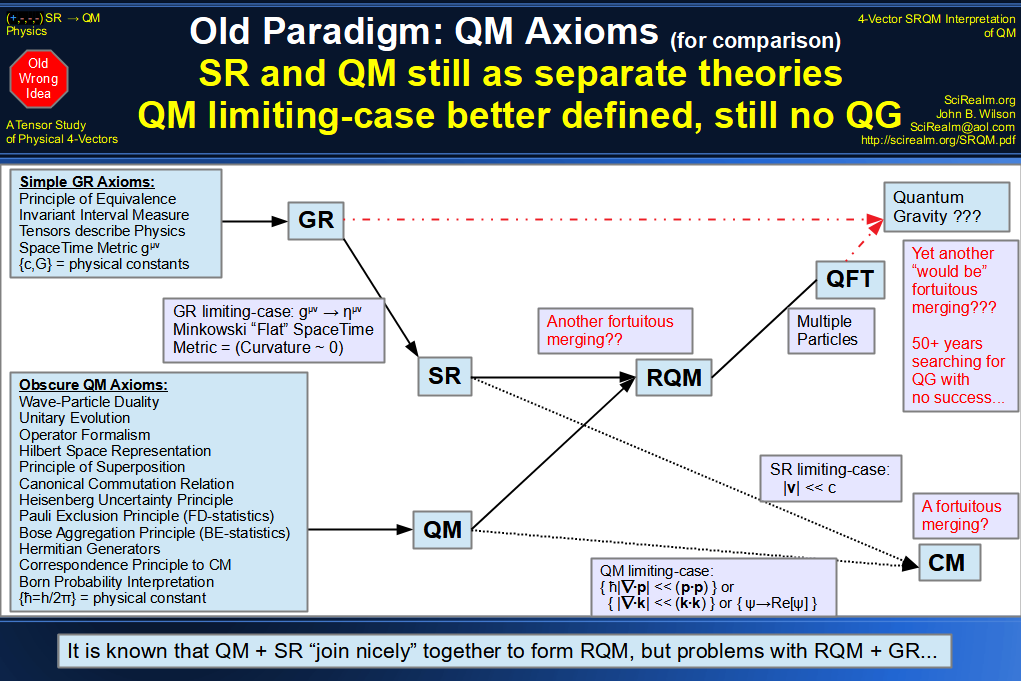### This is the new paradigm, based on the SRQM interpretation.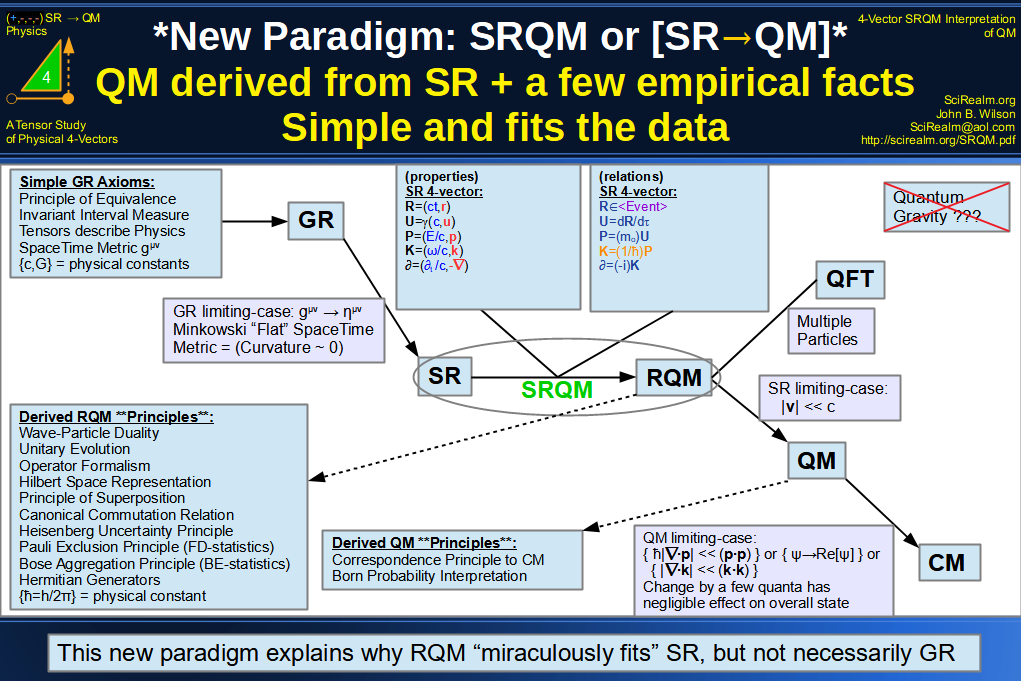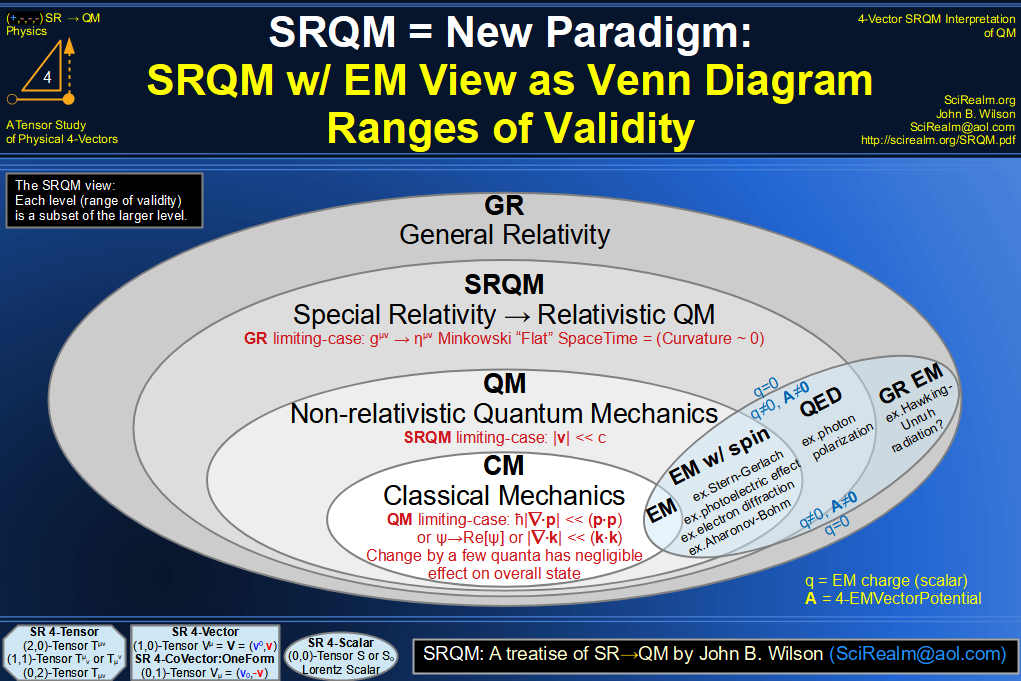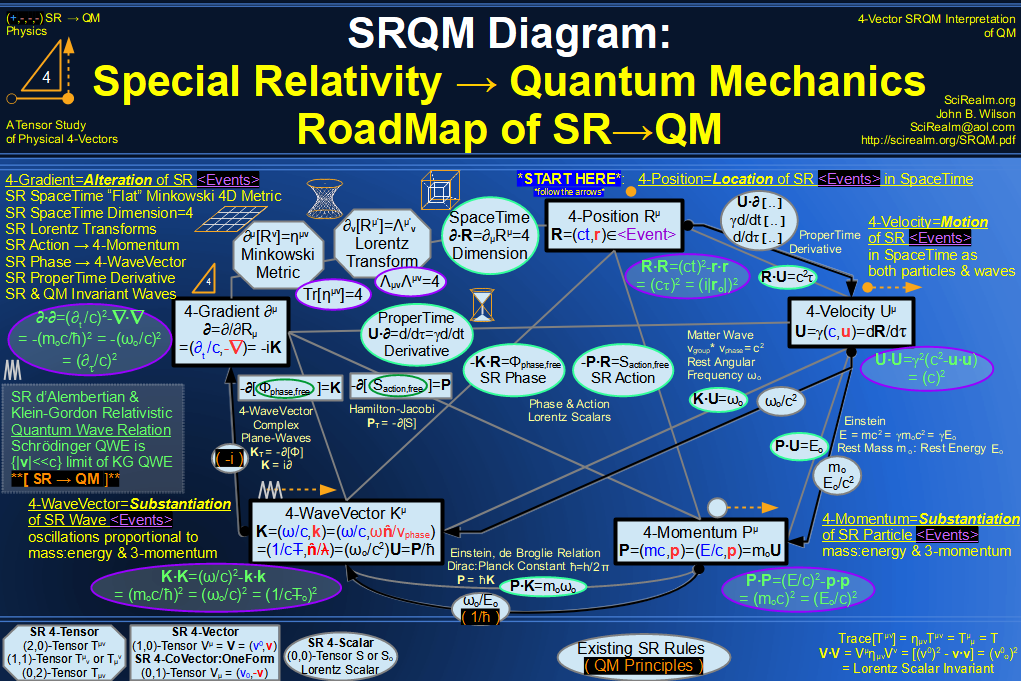=================================

Where do we start?
First:
I will show the really short version, the Roadmap from SR to QM...
Then:
I will begin with some basic assumptions and background stuff on Special Relativity (SR), Lorentz Scalars, 4-Vectors, and Tensors.
I will introduce the SR Physical 4-Vectors, which encapsulate the Physical Properties of Nature.
I will show how these SR 4-Vectors are related to one another in purely relativistic theory, i.e. the Physical Laws of Nature.
I will show a couple of relations that have previously been considered quantum assumptions/axioms/postulates,
but which are, in fact, fundamentally no different from the other SR relations.
I will derive relativistic quantum wave equations based just on these few SR relations.
I will derive the principles of Quantum Mechanics (QM), assuming no axioms of QM.

#### Roadmap from Special Relativity to Quantum Mechanics: (The Short Version)

4-Position R = (ct,r)
4-Velocity U = γ(c,u)
4-Momentum P = (E/c,p) = (mc,p)
4-WaveVector K = (ω/c,k)

Note the following relations between SR 4-Vectors:
U = dR/dτ
P = moU
K = (1/ћ)P = (ωo/c2)U
= -iK

Form a chain of SR Lorentz Invariant Scalar Equations, based on those relations:
R·R = (cτ)2
U·U = (c)2
P·P = (moc)2
= (Eo/c)2
K·K = (moc/ћ)2 = (ωo/c)2
· = (-imoc/ћ)2 = -(moc/ћ)2 = -(ωo/c)2

The last is a fundamental quantum relation.
When applied to a Lorentz Scalar, it gives the free particle Klein-Gordon Equation (the Relativistic Quantum Wave Equation for Spin-0 Particles).
When applied to a 4-Vector, it gives the free particle Proca equation (massive case mo > 0), and the free particle Maxwell equation (massless case mo = 0)
For the Maxwell equation, the fact that (mo = 0) is why you don't see the (ћ) in standard SR and EM.
The Schrödinger Equation, and hence Quantum Mechanics, is just the low-velocity (|v| << c) limiting-case of the KGE.
This is (RQM) = Relativistic Quantum Mechanics, derived from only:
--------------------------------------
5 of the Standard SR 4-Vectors.
4 really simple empirical relations between them.
1 SR rule for forming Lorentz Scalar Invariants, ie. the Minkowski Metric which gives the Lorentz Scalar Product.
--------------------------------------
As one of my physics professors (Dr. Valk - GA Tech Physics) used to say:
"Once you have the Schrödinger Equation, you have Quantum Mechanics."

I would modify this just a bit:
"Once you have the Klein-Gordon Equation, you have Relativistic Quantum Mechanics."
The Schrödinger Equation, and hence Quantum Mechanics, is just the low-velocity (|v| << c) limiting-case.
Likewise, Classical Mechanics is just the 'mixed wave' or 'non-phase aligned' limiting-case of Quantum Mechanics,
in which the divergence of a momentum state is very small compared to the magnitude-squared of the momentum state.
In other words, the limiting-case for which changing the state by a few quanta has a negligible effect on the overall state.
The Hamilton-Jacobi non-quantum limit {ћ|·p| << (p·p)} see Goldstein, Classical Mechanics, pg. 491

#### Basics

There are a couple of paradigm assumptions that we need to get clear from the start.
Relativistic stuff **IS NOT** the generalization of Classical stuff.
Classical stuff **IS** the low-velocity limiting-case approximation of Relativistic stuff { |v| << c }.
This includes Classical Mechanics and Classical QM (meaning the non-relativistic Schrödinger QM Equation).
Classical EM is for the most part already compatible with Special Relativity, although Classical EM didn't include intrinsic spin.
===
Along this same line of thought, SR Physical 4-Vectors *ARE NOT* generalizations of Classical or Quantum Physics 3-vectors.
While mathematically a Euclidean (n+1)D-vector is the generalization of a Euclidean (n)D-vector, the analogy ends there.
Minkowskian SR 4-Vectors *ARE* the primitive elements of 4D Minkowski SpaceTime.
Classical/Quantum Physics 3-vectors are just the spatial components of SR Physical 4-Vectors.
There is also a related Classical/Quantum Physics scalar which is just the temporal component of a given SR Physical 4-Vector.
The primary example is the 4-Position: (ct,x) → (ct,x,y,z)
These Classical/Quantum {scalar}+{3-vector} are the {temporal}+{spatial} "components" of an SR 4-Vector → (scalar, 3-vector).
However, different observers may see different "magnitudes" of the Classical/Quantum components from their point-of-view in SpaceTime,
while each will see the SAME actual SR 4-Vector at a given event in SpaceTime.
======
We will *NOT* be employing the commonly-(mis)used Newtonian classical limits {c→∞} and {ћ→0}.
Neither of these is a valid physical assumption, for the following reasons:

Both (c) and (ћ) are unchanging Physical Constants and Lorentz Invariants. Taking a limit where these change is nonphysical.
Many, many experiments verify that these constants have not changed over the lifetime of the universe.  They are CONSTANT.

Let E = pc. If c→∞, then E→∞. Then Classical EM light rays/waves have infinite energy.
Let E = ћω. If ћ→0, then E→0. Then Classical EM light rays/waves have zero energy.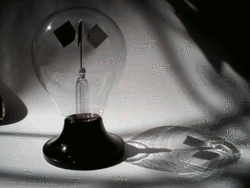Obviously neither of these is true in the Newtonian limit.
In Classical EM and Classical Mechanics, (c) remains a large but finite constant. Likewise (ћ) never becomes 0.
The correct way to take the limits is via:
The low-velocity non-relativistic limit {|v| << c}, which is a physically occurring situation.
The Hamilton-Jacobi non-quantum limit {ћ|·p| << (p·p)}, which is a physically occurring situation.
Alternately, and just to show that the Classical limit doesn't depend on the size of (ћ), this can be written as: {|·k| << (k·k)}
ie. when the divergence of the 3-wavevector is very small compared to the magnitude squared of the 3-wavevector
In other words, the limiting-case for which changing the state by a few quanta has a neglible effect on the overall state."
Also, I suspect that the covariant version is the actual way this should be written: {|·K| << (K·K)}
===
Furthermore, we will *NOT* be employing the commonly-used lazy convention of setting {c→1, ћ→1, G→1, etc.}.
While doing so "simplifies" many of the equations, one loses the power of dimensional and physical analysis.
Hey, these constants are an important aspect of nature and the physical universe.  Why ignore them?
Instead, it is always possible to design equations with new variables that "hide" extra dimensional constants if so desired.
In fact, I have followed online arguments which would be solved immediately if all the units/constants had been shown from the start.
It is confusing to non-experts, and even to experts, when the correct physical units/constants are not shown.
Don't be lazy when writing equations of the universe in the beginning and ending forms.
If you want to "hide" stuff in intermediate steps, then make it clear what you are doing.
It's damn hard enough just to get all the sign conventions right in SR and GR.
Now, to those saying go "Natural Units", where the constants are set to {1}...
The main argument that I have heard is "c = 3x108 m/s is a ridiculously large number to consider, so let's make the units c =1 distance unit/time unit"
Fine, set c = 1.  So, v = velocity = 1 = really fast.  Light travels one "light second" in one "second".  Wow - that is just so descriptive...  Is that a long way?
If I travel at unitless v = 1x10-8, with no seat belt, and hit something, will I probably die? How about if I travel at v = 1x10-7? 1x10-6?
The whole point of a measurement system is to make things relatable to us as humans.  The SI units do a pretty good job of this.
And yes, the speed of light (c) is pretty ridiculously huge on a human scale. Fact of nature. Deal with it.
We will not be using "Natural Units" here.  Save them for when you just want to do pure math or complex numerical computation, not physics.
Hiding universal constants from the fundamental physical equations of nature is just really dumb otherwise...
While the choice of a system of units is arbitrary, the fact that a universal constant exists is not:
Something travelling at { |v| = c } acts fundamentally differently than something travelling at { |v| < c }, the causality difference between light-like and time-like.
And writing it that way makes way more sense than writing it as { |v| = 1 } acts fundamentally differently than something travelling at { |v| < 1 }.
Likewise, we humans operationally experience time separations differently than space separations, where the difference factor is (c).  ΔX = (cΔt,Δx).
Again likewise, we operationally experience particle phenomena differently than wave phenomena, even though they are dual aspects for all known objects.
The difference factor is (ћ).
Something with a charge (q) acts differently in an EM field than something with no (q).  Etc, etc.
In short, don't be lazy. Show the dimensional factors in the main equations and when teaching physics.
You can set always them to unity later to simplify things when you run your numerical simulations... which is the only time that it is truly practical to do so...

#### References

Given that, let's begin:
Assume that Einstein 's General Relativity (GR) and the Mathematics of GR are essentially correct and our starting point.
See also: Intro to GR, Theoretical motivation for GR, Einstein's Genius (ScienceNews), General Covariance, Principle of Covariance,
Physical theories modified by general relativity,
Note: The LIGO gravitational wave observations are making "GR-as-a-good-starting-point" more solid with each new finding...
5 Binary BH mergers confirmed as of Nov 16, 2017
with each confirming GR {No dispersion, Speed of gravitational wave = speed of light, and GW170814 able to include the GR-predicted polarization states} :)
Announcement on Oct 3, 2017 - Nobel Prize Physics - Gravitational Wave Detection!!
And finally, the announcement of the Binary NS merger with optical counterpart, GW170817, the first of the gravitational multi-messenger observations.
*NEWS* Dec 03,2018 - Reanalysis of prior observing run data with better algorithms finds more GW signals - now we up to 10 BBH mergers.

Consider the SR [Low Mass = {Curvature ~ 0}] limiting-case of GR.
This is equivalent to a 4D SpaceTime being a pseudo-Riemannian manifold M with a metric gμν of signature (1,3),
and that for SR this metric gμν is restricted (taking a limiting-case)
to being the (flat SpaceTime) Minkowski metric ημν = Diag[+1,-1,-1,-1]{in Cartesian Form}

This gives Special Relativity (SR)Minkowski (Space /SpaceTime)Poincaré Invariance, which has the following properties:

The Principle of Relativity : The requirement that the equations describing the Laws of Physics have the same form in all admissible Frames of Reference
In other words, the Laws of Physics have the same form for all Inertial Observers
Mathematically this is Invariant Interval Measure ΔR·ΔR = (cΔt)2 - Δr·Δr = (cΔt)2 - |Δr|2 = Invariant
Speed of Light (c) = Invariant Lorentz Scalar = Physical Constant
The Principle of Covariance : The requirement that physical quantities must transform covariantly,
i.e. that their measurements in different frames of reference can be unambiguously correlated (via Lorentz Transformations ) - see the Covariance Group
Lorentz Invariance (Covariance) (for rotations and boosts {Aμ' = Λμ'νAν}: a Lorentz Transform on a 4-Vector in the group remains in the group)
Poincaré Invariance (Covariance) (for rotations, boosts, and translations {Aμ' = Λμ'νAν + ΔAν}), also known as full Relativistic Invariance
The Lorentz Gamma Factor is { γ = 1/√[1 - (v/c)2]  = 1/√[1 - β2] = dt/dτ}
Time Dilation {Δt' = γΔt}, Length Contraction {Δx' = Δx/γ}, Relativistic Mass {m = γmo}, Relativistic Momentum {p = γmov}, Relativistic Kinetic Energy {Ek = E - Eo = (γ-1)moc2)}
Minkowski Metric ημν = ημν = Diag[+1,-1,-1,-1]{in Cartesian Form}, with ηανηνβ = ηαβ = δαβ and Traceμν] = Tr[ημν] = ημνημν = δμμ = δνν = 4
ημν is the "Flat" SpaceTime Metric Tensor which is the low-mass, low-cuvature limiting-case of the GR Metric Tensor gμν
ημν has Metric Signature = (1,3,0), meaning counts of 1(+), 3(-), 0(null), which is also known as a Metric Signature Sum = -2 = (1) + (-3) = 1 positive + 3 negatives
which uses my preferred Metric Sign Convention (t0+) = {time: 0th-coordinate, positive(+), eal}, see 4-Vectors & Lorentz Scalars Reference for reasoning behind this
4D SpaceTime M is a Lorentzian manifold subclass of a pseudo-Riemannian manifold equipped with a metric g of signature (1,3,0),
allowing tangent vectors to be classified into time-like, space-like, and light-like (null) conditions, which supplies Causal Structure. See also Tangent Space.
Visualization is aided with the use of WorldLines and Minkowski Diagrams
Elements of Minkowski SpaceTime are Events (a temporal location {t}, a spatial location {x,y,z}) combined in equivalent dimensional form,
eg. (ct,x) → (ct,x,y,z) all components have dimension [length]
These elements are represented by 4-Vectors, which are actually Tensors, which allow the coordinate-free representations of Tensor Calculus/Ricci Calculus,
sometimes denoted as 4-Tensors {the index range {0..3} = 4}, or as (1,0)-Tensors, meaning {1 upper index, 0 lower indices}.
4-Vector notation: A  =  ... = (a0,a) = (a0,a1,a2,a3) → (at,ax,ay,az)
Tensor notation:  Aμ = (aμ) = (a0,ai) = (a0,a1,a2,a3) → (at,ax,ay,az)
4-Vectors can be used to represent Physical Properties, and relations (equations) between 4-Vectors represent Physical Laws = Laws of Science
ex. 4-Momentum P = (E/c,p) represents the physical properties of (temporal) Energy E and (spatial) 3-momentum p
{ P = moU = (Eo/c2)U} and { P·P = (E/c,p)·(E/c,p) = (E/c)2 - p·p = (Eo/c)2 = (moc)2} are physical laws.
see Invariant Mass, Mass in Special Relativity, Mass-Energy Equivalence
Scalar Products ( Inner products ) of 4-Vectors give Invariant Lorentz Scalars {(0,0)-Tensors} ex. A·B = AμημνBν = A'·B' = Scalar Invariant S
Tensor Products ( Outer Products ) of 4-Vectors give higher index tensors {(2,0)-Tensors, (3,0)-Tensors, etc.} ex. Fμν = (∂μAEMν - ∂νAEMμ)
Index raising and lowering, along with the previous operations, can be used to create tensors of mixed rank {(m,n)-Tensors} ex. ηανηνβ = δαβ
The Isometry Group (the set of all "distance-preserving" maps) of Minkowski SpaceTime is the Poincaré Group
Poincaré Group Symmetry : P = SO+(1,3) ⋉ 1,3(a non-Abelian Lie Group with 10 Generators )
Poincaré Generators  = { 3 rotations Ji + 3 boosts Ki + 1 time-translation P0 + 3 space-translations Pi, with i = 1..3}
Poincaré (10 "SpaceTime Generators") = Lorentz (6 "SpaceTime Rotation/Boosts" anti-symmetric Mμν) + Translations (4 "SpaceTime Translations" Pμ)
where the Lorentz Group Rotations mix orthogonal space directions; Lorentz Group Boosts mix time with a space direction
However, the double cover = SL(2,) ⋉ 1,3 = Spin(1,3) ⋉ 1,3 is the total Symmetry Group of Minkowski SpaceTime :
i.e. the connected double cover of the Poincaré Group, as this includes particle symmetries and the ability to describe spin 1/2 fields.
SL(2,) is the group of complex (2x2) matrices with unit determinant.
New X'μ  = 4-Tensor Antisymmetric
Lorentz Transform Mμν
Rotations j = Mab
Boosts k = M0b = -Mb0
3 + 3 = 6
Original Xμ  + 4-Vector
SpaceTime Translation ΔXμ ~ Pμ
Time Translation H = P0
Space Translation p = Pi
1 + 3 = 4
 X'0 X'1 X'2 X'3
=
 M01 M02 M03 M10 M12 M13 M20 M21 M23 M30 M31 M32
 X0 X1 X2 X3
+
 ΔX0 ΔX1 ΔX2 ΔX3
Total of 6 + 4 = 10 parameters
Poincaré Transform = Lorentz Transform + SpaceTime Translation
X'μ = Mμν Xν + ΔXμ
where colors indicate
 temporal spatial mixed
The (10) one-parameter groups can be expressed directly as exponentials of the generators:
U[I, (0,λ)] = e^(-iλ·p): (3) Linear Momentum p
U[I, (a0,0)] = e^(ia0·H) = e^(ia0·P0): (1) Hamiltonian = Energy = Temporal Momentum H
U[Λ(iλθ̂/2), 0] = e^(iλθ̂·j): (3) Angular Momentum j
U[Λ(λφ̂/2), 0] = e^(iλφ̂·k): (3) Rotationless Boost k
The Poincaré Algebra is the Lie Algebra of the Poincaré Group :
============
Covariant form: P = Generator of Translations, M = Generator of Lorentz Transformations (Rotations & Boosts)
These are the commutators of the the Poincaré Algebra :
[Xμ, Xν] = 0μν
[Pμ, Pν] = -iћq(Fμν) if interacting with EM field; otherwise = 0μν for free particles
Mμν = (XμPν - XνPμ) = iћ(Xμν - Xνμ)
[Mμν, Pρ] = iћ( ηρνPμ - ηρμPν)
[Mμν, Mρσ] = iћ(ηνρMμσ + ημσMνρ + ησνMρμ + ηρμMσν)

 Translational Operator ∂μ Kμ Pμ Equivalent = ∂μ = i∂μ = iћ∂μ Normal Commutator [∂μ, Xν] = ημν [Kμ,Xν] = iημν [Pμ, Xν] = iћημν Reversed Commutator [Xν, ∂μ] = -ημν [Xν, Kμ] = -iημν [Xν, Pμ] = -iћημν

 Rotational Momentum Operator M Mμν Mμν Mμν Dimensionless Rotational Operator O Oμν Oμν Oμν Equivalent = iћ(Xμ∂ν - Xν∂μ) = ћ(XμKν - XνKμ) def. = (XμPν - XνPμ) Equivalent def. = (Xμ∂ν - Xν∂μ) = (1/i)(XμKν - XνKμ) = (1/iћ)(XμPν - XνPμ) Normal Commutator [Mμν, ∂ρ] = iћ(ηρν∂μ - ηρμ∂ν) [Mμν, Kρ] = iћ(ηρνKμ - ηρμKν) [Mμν, Pρ] = iћ(ηρνPμ - ηρμPν) Normal Commutator [Oμν, ∂ρ] = (ηρν∂μ - ηρμ∂ν) [Oμν, Kρ] = (1/i)(ηρνKμ - ηρμKν) [Oμν, Pρ] = (1/iћ)(ηρνPμ - ηρμPν) Reversed Commutator [∂ρ, Mμν] = -iћ(ηρν∂μ - ηρμ∂ν) = iћ(ηρμ∂ν - ηρν∂μ) [Kρ, Mμν] = -iћ(ηρνKμ - ηρμKν) = iћ(ηρμKν - ηρνKμ) [Pρ, Mμν] = -iћ(ηρνPμ - ηρμPν) = iћ(ηρμPν - ηρνPμ) Reversed Commutator [∂ρ, Oμν] = -(ηρν∂μ - ηρμ∂ν) = (ηρμ∂ν - ηρν∂μ) [Kρ, Oμν] = -(1/i)(ηρνKμ - ηρμKν) = (1/i)(ηρμKν - ηρνKμ) [Pρ, Oμν] = -(1/iћ)(ηρνPμ - ηρμPν) = (1/iћ)(ηρμPν - ηρνPμ)

============
Component form: Rotations Ji = -εimnMmn/2, Boosts Ki = Mi0
[Jm,Pn] = iεmnkPk
[Jm,P0] = 0
[Kj,Pk] = iηjkP0
[Kj,P0] = -iPj
[Jm,Jn] = iεmnkJk
[Jm,Kn] = iεmnkKk
[Km,Kn] = -iεmnkJk, a Wigner Rotation resulting from consecutive boosts
[Jm + iKm,Jn - iKn] = 0
============
Poincaré Algebra has 2 Casimir Invariants = Operators that commute with all of the Poincaré Generators
These are {P2 = PμPμ = (moc)2, W2 = WμWμ = -(moc)2j(j + 1) }, with Wμ = (-1/2)εμνρσJνρPσ as the Pauli-Lubanski Pseudovector
Alt Def: Wμ = (1/2)εμνσρPνMσρ = (1/2)εμνσρMσρPν
[P2,P0] = [P2,Pi] = [P2,Ji] = [P2,Ki] = 0: Hence the 4-Momentum Magnitude squared commutes with all Poincaré Generators
[W2,P0] = [W2,Pi] = [W2,Ji] = [W2,Ki] = 0: Hence the 4-SpinMomentum Magnitude squared commutes with all Poincaré Generators
Furthermore:
[Pμ,Pν] = 0
[Wμ,Pν] = 0
(1/i)[Wμ,Mσρ] = δμσWρ - δμρWσ
(1/i)[Wμ,Wν] = εμνσρPσWρ

****
Very importantly, the Poincaré group has Casimir Invariant Eigenvalues  = { Mass m, Spin j },
hence Mass *and* Spin are purely SR phenomena, no QM axioms required!
****

This Representation of the Poincaré Group or Representation of the Lorentz Group
is known as Wigner's Classification in Representation Theory of Particle Physics
Apparently still true in GR is Infinitesimal Invariant Interval Measure dR·dR = (cdτ)2 = (cdt)2 - dr·dr = (cdt)2 - |dr|2

List of mathematical topics in Relativity
The Wightman Axioms
Wigner's Theorem
Covariant Formulation of Classical Electromagnetism
Invariance Mechanics
SpaceTime Symmetries
SpaceTime Algebra

#### Justification of the SRQM treatise

Given that, let's begin:
Justification of the SRQM treatise (GR→limiting-case→SR→RQM→limiting-case→QM→limiting-case→CM) comes from the following:

General Relativity:
Tests of General Relativity,
(Mercury Perihelion Precession, Gravitational Light Deflection/Lensing, Gravitational Redshift, Shapiro Effect Light Delay, EEP, Geodetic and Frame-dragging Effects)
(Metric Coupling from Solar System Tests, Physical Constants, Time Invariance of Physical Constants)
(Isotropy from Quadrupolar Shift of Nuclear Energy Levels)
(Universality of Freefall from Lunar Laser Ranging and Orbiting Gyroscopes)
(Gravitational Redshift from Spaceborne Clocks)
(Structure of the Metric from Planetary Perihelion Shift)
(Lorenz Gauge Condition and Inhomogeneous Wave Eqn from Gravitational Waves)
(Mass Equivalence, Gravitational Time Dilation, Precession of Orbits, Thomas Precession, de Sitter Precession, Lense-Thirring Precession, Orbital Decay via Gravitaional Waves, Strong Field Effects)
Relativistic Geodesy, For The First Time, a Portable Atomic Clock Has Been Used to Measure Gravity,
(Weak Equivalence Principle = WEP = Equality of Acceleration of Test Bodies = Universality of Free Fall<10-16)
(Einstein Equivalence Principle <10-14)
(Strong Equivalence Principle)
(Local Lorentz Invariance <10-20)
(Local Position Invariance <10-6)
(No gravitational wave dispersion, Speed of gravitational wave = speed of light, and GW170814 able to include the GR-predicted polarization states)
Testing Einstein's Universe, General Relativity and Cosmology Overview,
Precision Tests of General Relativity in Space,
Gravity Probe A, Gravity Probe B, the Laser Relativity Satellite (LARES),
MICROSCOPE (satellite), STEP (satellite),
Gravitational Waves (LIGO observations), GW170814, GW170817, List of GW observations,
Binary/Triple pulsars:
Hulse-Taylor binary PSR B1913 + 16,
Double pulsar PSR J0737-3039,
Binary (neutron star/white dwarf) PSR J0348 + 0432,
Millisecond Pulsar (+2 close orbit stars) PSR J0337+1715,
The relativistic pulsar-white dwarf binary PSR J1738+0333 II. The most stringent test of scalar-tensor gravity,
Highly Relativistic Double Neutron Star Binary PSR J1946+2052 (1.88hr orbit),
Trinary: PSR J0337+1715,
Einstein Rings, Einstein Cross,
Experimental Tests of Graviational Theory (pdf), Experimental Tests of General Relativity (UFlorida),
Tests of General Relativity: A Review (2017 pdf),
Tests of General Relativity with GW170817,
Relativity's Long String of Successful Predictions,
Eötvös experiment, The Eöt-Wash Group: Laboratory Tests of Gravitational and sub-Gravitational Physics,
Einstein's general relativity passes another stringent test (Cosmological Redshift Jul 2016),
A Picture-Perfect Solar Eclipse Experiment: Gravitational Light Deflection (Feb 2018),
Signal Timing Tests (Cassini Saturn Probe),
General relativity caught in action around black hole: Wobbling disk of orbiting debris shows yanking and twisting of spacetime (Dec 2015),
Trio of dead stars upholds a key part of Einstein’s theory of gravity (Jan 2018) Strong Equivalence Principle for UltraDense objects,
Even This Crazy System of Ultradense Dead Stars Can't Break General Relativity: Einstein. Right. Again. (Jan 2018),
Theory of general relativity proven yet again in new research (Jul 2018) PSR J0337+1715, Delta value <3x10-6, GR says 0
S0-2, Star Close-Orbiting the Super-Massive Black Hole Sagittarius A*, Set for closest approach in mid-2018, I bet here/now (early 2018) that it makes no conflicting observations with GR
Milky Way's Black Hole Provides Long-Sought Test of Einstein's General Relativity (July 26, 2018), Confirms GR, as predicted...   :) !
Star S2 orbiting Sagittarius A supermassive black hole proves Einstein relativity first direct observation,
A new Einstein cross is discovered (Mar 2019),
Equivalence principle holds up inside an orbiting satellite (MICROSCOPE Dec 2017),
Einstein equivalence principle survives quantum test, acceleration applies even for atoms in superposition of energy states (Apr 2017),
Quantum test of the equivalence principle for atoms in superpositions of internal energy eigenstates (Apr 2017),
Cryogenic test probes Einstein's equivalence principle, general relativity, and SpaceTime 'foam' - Local Position Invariance (Jan 2017),
Scientists Check Einstein's Predictions Using Earth Itself As The Laboratory (Jun 2018),
NIST atomic clock comparison confirms key assumptions of 'Einstein's elevator' (Jun 2018), LPI β parameter measured to (2.2 ± 2.5)x10-7: GR predicts 0
A null test of general relativity based on a long-term comparison of atomic transition frequencies (Jun 2018),
A null test of General Relativity: New limits on Local Position Invariance and the variation of fundamental constants (Feb 2018),
Einstein's theory of gravity holds – even in extreme conditions (Jun 2018),
Einstein’s general relativity reigns supreme, even on a galactic scale (Jun 2018),
Einstein Wins Again! General Relativity Passes Its First Extragalactic Test (Jun 2018), Potential Ratio γ parameter measured to (0.97 ± 0.09): GR predicts 1
this extends the realm of validity of GR from the Solar-System-sized tests to at least 7000 light years...
Einstein’s general theory of relativity passes a supermassive test (Mar, 2019), GRAVITY team measures H and He absorption lines in grav field 10^6 x Earth and 10x WhiteDwarf observations
Quantum Foam - See Constraints and Limits (Wikipedia),
INTEGRAL observatory puts limits on Quantum Foam idea of SpaceTime (June 2011),
NASA telescopes set limits on SpaceTime Quantum "Foam" (May 2015),
One particle’s trek suggests that ‘spacetime foam’ doesn’t slow neutrinos (Jul 2018),
Fermilab Holometer rules out pixelated SpaceTime (Dec 2015), No Holographic Noise (Apr 2016), (SpaceTime not Quantized at the Planck Scale)
Is a tabletop search for Planck scale signals feasible? (Dec 2012), (send single photons through an ultracold crystal and see if block displacements are in discrete jumps)
Test of equivalence principle searches for effects of spin-gravity coupling (Jul 2014),
Tests of Equivalence Principle (wiki),
Tests of the Weak Equivalence Principle (2014),
The Confrontation between General Relativity and Experiments (2014), Was Einstein Right? Testing Relativity at the Centenary,
Stronger tests of Einstein's theory of general relativity with binary neutron stars,
Relativity Gets Thorough Vetting from LIGO (May 2016)***,
Bounding the speed of gravity (Nov 2017),
Star orbits around the supermassive Black Hole at Milky Way center Sagittarius A* (Aug 2017),
Deep Galaxy Map (May 2016), Clumped Galaxies (Jun 2014),
General Relativity and Cosmology: Unsolved Questions and Future Directions (Sep 2016),
Putting Relativity to the Test (PBS Nova),
Black Hole No-Hair Theorem, Testing GR "No-Hair" Theorems, Seeking proof for the no-hair theorem (2014),
GW detection: properties consistent with the black hole no-hair theorem,
Curtains down for the black hole firewall paradox: Making gravity safe for Einstein again (2013)(QM conforms to GR),
Superconducting-Gravimeter Tests of Local Lorentz Invariance (Dec 2016),
Relativistic Geodesy - JILA/NIST strontium clocks (2015),
Relativistic Geodesy - NOAA Report,
Mathematicians propose alternative explanation for cosmic acceleration, based on original GR, {Friedmann is unstable solution} (Dec 2017),
Einstein’s general relativity reveals new quirk of Mercury’s orbit (Apr 2018),
How gravitational waves could solve some of the Universe’s deepest mysteries (Apr 2018),
Three gas clouds nearly grazed the edge of the Milky Way’s black hole, The observations confirm that the supermassive object really is a {GR} black hole (Oct 2018),
Physicists Used Einstein's Relativity To Successfully Predict A Supernova Explosion (Nov 2018),
Synopsis: Satellite Mishap Provides Chance for Relativity Test (Dec 2018),
Historic First Images of a Black Hole Show Einstein Was Right (Again) (Apr 10 2019),
Tidal forces are gravitational waves (Arxiv Dec 2, 2019),

Special Relativity:
Tests of Special Relativity (Wikipedia), Tests of Special Relativity (BaezPhysics), Experimental Support for Einstein's Theory,
Time Dilation, Length Contraction, Lorentz Covariance, Speed of Light Constant (c),
Modern searches for Lorentz Violation (none confirmed to date, and some constraints up to 10^-34 level), in Antimatter, in Neutrino Oscillations, in Electrodynamics,
Standard Model Extension - Looking for Lorentz Violations
Searches for Lorentz and CPT Violations, Data Tables for Lorentz and CPT Violations,
Modern Tests of Lorentz Invariance (2005),
Viewpoint: Catching relativity violations with atoms (Jul 2009),
Still no violation of Lorentz Symmetry, despite strongest test yet (Dec 2016),
Separate experiments show no evidence of violation of Lorentz invariance (Nov 2017),
Right Again, Einstein: Special Relativity Works Even in Ghostly High-Energy Neutrinos (Lorentz Invariance)(July 2018),
Limits on Neutrino Lorentz Violation from Multimessenger Observations of TXS 0506+056 (Jul 2018),
Synopsis: Relativity Survives Scrutiny, Again (Nov 2017),
Lorentz Symmetry (non-)Violations from Matter-Gravity Couplings with Lunar Laser Ranging (Nov 2017),
Most precise test of Lorentz symmetry for the photon finds that the speed of light (c) is indeed constant (Sept 2015),
Michelson-Morley experiment for electrons: Quantum-information techniques used to explore fundamental physics (Feb 2015),
Atomic clocks make best measurement yet of relativity of time (Mar 2017),
Quantum gas atomic clocks measure time (Oct 2017),
Ultraprecise atomic clocks put Einstein's special relativity to the test (Mar 2019),
Quantum computer as detector shows space is not squeezed (Jan 2015),
Physicists Precisely Measure Proton’s Magnetic Moment - Supporting CPT Invariance (Nov 2017),
At tremendous precision, the proton and antiproton still seem identical - Supporting CPT Invariance (Oct 2017),
Stringent Tests of CPT Invariance,
Tests of local position invariance using continuously running atomic clocks (Jan 2013),
Global Positioning System Test of the Local Position Invariance of Planck’s Constant (Mar 2012),
Tests of Relativistic Energy and Momentum
Hughes-Drever Experiment
Test Theories of Special Relativity
Parameterized_post-Newtonian_formalism
Quantum Experiment Helps Prove Einstein's Theory of Relativity,
Three Experiments That Show Relativity Is Real (Jul 2015),

To date, SR and GR are compatible with all known physical experiments and observations.
Basically, all known parameterized Alternatives to General Relativity (i.e. GR + stuff) are being forced to match GR (stuff → 0), based on very stringent testing.

Likewise, QM and QED are confirmed by thousands of different experiments.
Precision tests of QED
Experimental checks on the photon mass
History of QM
Theoretical and experimental justification for the Schrödinger equation
Synopsis: Cosmic Test of Quantum Mechanics (Feb 2017),
Team invents new way to 'see' the quantum world (Mar 2018),
Three Experiments That Show Quantum Physics Is Real (Jul 2015),
Quantum Field Theory in Curved SpaceTime,

However, some cracks in QM are starting to show...
A Rare Element From The Edge of The Periodic Table Is Breaking Quantum Mechanics (Oct 2017),
Breaking the rules: Heavy chemical elements alter theory of quantum mechanics (Oct 2017),
Viewpoint: Heaviest Element Has Unusual Shell Structure: significant impact of relativistic effects upon the shell structure (Jan 2018),
Relativistic effects on long-range interactions between objects (Mar 2018),
Quantum theory: Einstein saves the quantum cat, Universal decoherence due to gravitational time dilation,
Relativistic Quantum Chemistry,
Can LIGO test Quantum Gravity?,
Will Quantum Mechanics Swallow Relativity? (my answer - No!, quite the opposite, see Holometer results for example),
The Casimir Effect (wiki - see section on Relativistic van der Waals force),
The Casimir Effect and the Quantum Vacuum (Jaffe 2005),
--In discussions of the cosmological constant, the Casimir effect is often invoked as decisive evidence that the zero point energies of quantum fields are "real''. On the contrary, Casimir effects can be formulated and Casimir forces can be computed without reference to zero point energies. They are relativistic, quantum forces between charges and currents. The Casimir force (per unit area) between parallel plates vanishes as α, the fine structure constant, goes to zero, and the standard result, which appears to be independent of α, corresponds to the α→∞ limit,
Proof that Casimir force does not originate from vacuum energy,
1975: The year that quantum mechanics met gravity (COW experiments - Gravitationally induced quantum interference),
Gravitation and quantum interference experiments with neutrons (2012),
Gravity Neutron Interferometry,
Gravitationally induced quantum interference using a floating interferometer crystal (Nov 2006),
Neutron interference in the Earth’s gravitational field - relativistic derivation {general relativity has an application in quantum physics}(Jan 2017),
The Relativistic Quantum Double-Slit Experiment,
Viewpoint: Measuring the Tidal Force on a Particle’s Matter Wave (May 2017),
Viewpoint: Neutrons Knock at the Cosmic Door (Apr 2014),
Gravity doesn’t care about quantum spin (Jul 2016),
Quantum Test of the Universality of Free Fall (Jun 2014),
Key Einstein principle survives quantum test: Equivalence of gravity, acceleration applies even for atoms in superposition of energy states (Apr 2017),
Quantum test of the equivalence principle for atoms in superpositions of internal energy eigenstates (Apr 2017),
Synopsis: Free Falling Matter Waves (May 2014),
Synopsis: Skydiving Spins (July 2016),
Synopsis: A Test of Gravity’s Quantum Side (Dec 2017),
Tests of the Gravitational Inverse-Square Law below the Dark-Energy Length Scale (Nov 2006),
qBounce, the Quantum Bouncing Ball Experiment (Dec 2011),
A Gravity of Earth Measurement with a qBOUNCE Experiment (Dec 2015),
Quantum Gravitational Spectroscopy - GRANIT (2015),
Gravitationally induced phase shift on a single photon (Dec 2016),
A Tabletop Experiment For Quantum Gravity (Nov 2017), A Spin Entanglement Witness for Quantum Gravity (Jul 2017),
Gravity Resonance Spectroscopy Constrains Dark Energy and Dark Matter Scenarios (Apr 2014),
Interferometry with Bose-Einstein Condensates in Microgravity (Jan 2013),
Physicists Have Detected a Friction-Like Force in a Perfect Vacuum {Without breaking the fundamental laws of physics}(Feb 2017),
Relational interpretation of the wave function and a possible way around Bell's theorem (Feb 2006),
Curtains down for the black hole firewall paradox: Making gravity safe for Einstein again (2013)(QM conforms to GR),
Quantum probability assignment limited by relativistic causality (2016),
In Search For The True Relationship Between General Relativity And Quantum Mechanics (Jan 2018),
Finding Solutions To Contradictions In Relativity And Quantum Mechanics (Feb 2018),
Quantum Locality, Rings a Bell?: Bell's inequality meets local reality and true determinism (Nov 2017),
The Puzzle of Quantum Reality (Mar 2018),
Atoms and antiatoms haven’t crashed Albert Einstein’s theory of relativity—yet (Apr 2018),
Are Space And Time Quantized? Maybe Not, Says Science (Jun 2018), (not in any way that alters GR I say),
Is time quantized? In other words, is there a fundamental unit of time that could not be divided into a briefer unit?,(Baez and Unruh opine),
Searching for errors in the quantum world (Sept 2018),
Reimagining of Schroedinger's Cat Breaks Quantum Mechanics ... and Stumps Physicists (Sept 2018),
The point here, is that quantum effects do not appear to alter GR physics, but GR can alter QM physics.
Quantum properties do not alter GR's universality of free-fall, SR's {E=mc2}, etc.
GR gravity can induce quantum interference in cold neutron wavefunctions, etc.
...which is what you should expect based on the SRQM paradigm of (GR→limiting-case→SR→RQM→limiting-case→QM→limiting-case→CM)...

Likewise, a lot of "Alternative Physics" theories are being severely constrained.
Tabletop experiment puts new limits on string theory (Jan 2007),
Gravity doesn’t leak into large, hidden dimensions: Observations from neutron star smashup challenge some theories that include unknown realms (Feb 2018),
New gravitational wave detection with optical counterpart rules out some dark matter alternatives, GW170817 Falsifies Dark Matter Emulators,
Dark Matter Winners And Losers In The Aftermath Of LIGO (Dec 2017),
Dark Energy Winners And Losers In The Aftermath Of LIGO (Dec 2017),
Viewpoint: Reining in Alternative Gravity (Dec 2017),
Why Einstein's Elegant Theory Of Relativity Has Stood The Test Of Time (Dec 2016),
What Astronomers Wish Everyone Knew About Dark Matter And Dark Energy (Apr 2018),
Looking for "Nothing" to Test Gravity (Feb 2018),
To Test Einstein's Equations, Poke a Black Hole: Progress on Black Hole Stability Conjecture (Mar 2018),
Only Dark Matter (And Not Modified Gravity) Can Explain The Universe (Mar 2018),
No quantum gravity signature from the furthest quasars (Aug 2011),
Troubled Times for Alternatives to Einstein’s Theory of Gravity (Apr 2018),
Why String Theory Is Still Not Even Wrong (Apr 2017),
Pulsar Limits “Fifth Force” Interactions with Dark Matter (Jun 2018),
Measurement Analysis and Quantum Gravity: Justification for ...QG... must be based on empirical tests and does not follow from logical arguments alone, Aug (2008),
Study finds flaws in emergent gravity (Aug 2018),
Exclusion of standard ℏω gravitons by LIGO observation (Aug 2018),
Modified Gravity Could Soon Be Ruled Out, Says New Research On Dwarf Galaxies (Aug 2018),
Gravitational waves provide dose of reality about extra dimensions (Sep 2018),
Exclusion of standard ℏω gravitons by LIGO observation (Aug 2018),
String theory: Is dark energy even allowed? (Oct 2018),
String theory is said to be fundamentally incompatible with our current understanding of 'dark energy'.
Cumrun Vafa's conjecture, which prohibits certain types of fields, would also prohibit things that we already know to exist,
The Higgs field has properties that should actually be forbidden by Vafa's string-theoretic conjecture -- and the Higgs field is considered an experimentally proven fact.
Analysis by John:
"I have said it before, GR is *not* wrong. - String Theory is wrong.
There is no experimental evidence of extra dimensions.
There is no evidence of Lorentz Invariance violations.
Experimental evidence still indicates point-like particle interactions.
There is so far no evidence of super-symmetric particles.
There is evidence for dark energy, with GR's cosmological constant matching the data pretty well.
And now there is a property of String Theory that is incompatible with the Higgs particle, which has experimental confirmation."
Our Universe's Gravity Isn't Leaking Into Other Dimensions, Physicists Find (Nov 2018),

And for the future:
Semiclassical Gravity: The approximation to the theory of quantum gravity in which one treats matter fields as being quantum and the gravitational field as classical (wiki),
Study finds semiclassical gravity counterintuitive, but on the horizon of testability (May 2013),
Asymptotic safety in quantum gravity (wiki),
What Are Quantum Gravity's Alternatives To String Theory? (Dec 2015),
Fundamental quantum optics experiments conceivable with satellites — reaching relativistic distances and velocities,
Is Quantum Gravity Necessary? (1) - I say no!
Is Quantum Gravity Necessary? (2) - I say no!
Is Gravity Quantum? (SciAm Aug 2018), - I say no!
How We'll Finally Wind Up Testing Quantum Gravity (Apr 2016),
Gravitational Waves Will Show (or not) The Quantum Nature Of Reality (Mar 2016),
A blueprint for a simultaneous test of quantum mechanics and general relativity in a space-based quantum optics experiment (Nov 2016),
We Now Have Atomic Clocks So Precise, They Could Detect Space-Time Distortion  (Nov 2018),
Measurement of the Gravity-Field Curvature by Atom Interferometry
Synopsis: Black Hole Test for Gravity
Our universe has antimatter partner on the other side of the Big Bang, say physicists
CPT-Symmetric Universe

News Topic Searches:
General Relativity News,
Special Relativity News,
Relativity Tests News,
Relativity Theory Tests News,
Tabletop Relativity News,
Relativistic News,
Relativistic Geodesy News,
CPT Symmetry News,
CPT Invarinace News,
Poincaré Symmetry News,
Poincaré Invariance News,
Lorentz Invariance News,
Local Invariance News,
LLI Invariance News,
LPI Invariance News,
Equivalence Principle News,
Einstein Theory News,
LIGO News,
Black Hole News,
Gravitational Wave News,
Semiclassical Gravity News,
Quantum Gravity News,
Quantum Relativity News,
SpaceTime News,
Fundamental Constants News,
Physical Constants News,
Gravity Interferometry News,
Relativity Interferometry News,
Quantum Entanglement News,

Universal physical constants that are Lorentz Scalars (with experimental verification of the Non-time-variation of fundamental constants, Baez website constants, Baez website variability,):
Speed of Light (c)Measurement of (c)
Planck Constant (h) and reduced Planck Constant (ћ): with { ћ = h/2π }, Measurement of (h)
EM Elementary Charge (e), Measurement of (e)
Electric constant = Vacuum Permittivity (εo), Magnetic constant = Vacuum Permeability (μo): Really just one independent constant due to { εoμo = 1/c2 }
Gravitational Constant (G)
Electron Rest Mass (me)
Boltzmann Constant (k = kB)

(c) = Sqrt[U∙U]
(ћ) = [P∙U]/[K∙U]
Fαν = (1/q)F
∂·Fαν = (μo)J = (1/c2εo)J
(mo) = [P∙U]/[U∙U]
Gμν + Λgμν = (8πG/c4)Tμν with Gμν = Rμν - (R/2)gμν

Distant Quasars Show That Fundamental Constants Never Change (Jan 2017),
Synopsis: Pinpointing Planck’s Constant with GPS (Mar 2012),
Viewpoint: Time Trials for Fundamental Constants (Nov 2014),

Fine Structure Constant { α = (1/4πεo)(e2/ћc) = o/4π)(ce2/ћ) },
Gravitational Coupling Constatnt { αG = Gme2/ћc },
Note that these are made up from the Lorentz Scalar constants from above...

Mathematician Emmy Noether changed the face of physics, linking two important concepts in physics: conservation laws and symmetries

Now, just to give some more background on where this is all heading:

Special Relativity (SR) and Quantum Mechanics (QM) are completely compatible in Relativistic Quantum Mechanics (RQM)
The combination of both theories leads to Relativistic Wave Equations (RWE),
The relativistic formulation is more successful than the original "classical" quantum mechanics:
The prediction of antimatter, electron spin, spin magnetic moments of elementary spin-1/2 fermions, fine structure, and quantum dynamics of charged particles in electromagnetic fields.
The most basic of the RQM equations (spin 0, massive) is the Klein-Gordon Equation
The next level RQM equation (spin 1/2, massive) is the Dirac Equation
And the next RQM equation (spin 1, massless) is the Maxwell Equation, which is actually standard EM
The formalism developed then leads smoothly into Quantum Field Theory (QFT) and Gauge Theory via Second Quantization (multiple particles = field quantization).
Examples of these are: Quantum Electrodynamics (QED) and Yang-Mills Theory and The Standard Model

RQM is required for various theorems:
Spin-Statistics Theorem, CPT Theorem, Coleman-Mandula Theorem, Haag's Theorem, Weinberg-Witten Theorem, etc.
Various QM phenomena are derivable once you have RQM:
Quantum Superposition, Pauli Exclusion Principle, Canonical Commutation Relation, Heisenberg Uncertainty, etc.
Newtonian Limit of the (spin 0) RQM Klein-Gordon Equation is the QM Schrödinger Equation (First Quantization)
Newtonian Limit of the (spin 1/2) RQM Dirac Equation is the QM Pauli Equation

These are the free particle versions of the listed equations (no interaction with a potential)
All based on the fundamental equation we derived in the Roadmap above.
· = (-imoc/ћ)2 = -(moc/ћ)2 = -(ωo/c)2
 Standard Model Elementary Particle Relativistic Wave Equations (RWE) Relativistic Wave Equations (RWE) Newtonian Limit ( |v| << c ) Particle Type Spin Statistics Field RQM Massless (mo = 0) RQM Massive (mo > 0) QM Massive (mo > 0) Fundamental 0 Boson Lorentz Scalar ψ Scalar Wave (∂·∂)ψ = 0 Klein-Gordon Equation (∂·∂ + (moc/ћ)2)ψ = 0 Schrödinger Equation (iħ∂t)ψ ~ [(moc2) - (ħ∇)2/2mo]ψ Fundamental 1/2 Fermion Spinor Ψ Weyl Equation [(iγμ∂μ)]Ψ = 0 → [(σμ∂μ)]Ψ = 0 Dirac Equation, Majorana Equation [(iγμ∂μ) - (moc/ћ)]Ψ = 0 (ΓμPμ)Ψ = (moc)Ψ iћ(Γμ∂μ)Ψ = (moc)Ψ Pauli Equation (iħ∂t)Ψ ~ [(moc2) + (σ·p)2/2mo]Ψ Fundamental 1 Boson 4-Vector A Maxwell Equation (∂·∂)A = 0 Proca Equation (∂·∂ + (moc/ћ)2)A = 0 ? Composites 3/2 Fermion Spinor-Vector Majorana Rarita-Schwinger Rarita-Schwinger Equation ?? 2 Boson (2,0)-Tensor Graviton??

Each of these can be free particle, particle with source, or particle with QM source:
eg.
(·)Aν = 0ν: The Free Classical Maxwell EM Equation {no source, no spin effects}
(·)Aν = μoJν: The Classical Maxwell EM  Equation {with 4-Current J source, no spin effects}
(·)Aν = q(ψ̅ γν ψ): The QED Maxwell EM Spin-1 Equation {with QED source, including spin effects}

These are the minimal-coupled versions of the listed equations (interaction with an EM potential)
 Standard Model Elementary Particle Relativistic Wave Equations (RWE) Relativistic Wave Equations (RWE) Newtonian Limit ( |v| << c ) Particle Type Spin Statistics Field RQM Massless (mo = 0) RQM Massive (mo > 0) QM Massive (mo > 0) Fundamental 0 Boson Lorentz Scalar ψ Scalar Wave (D·D)ψ = 0 Klein-Gordon Equation (D·D + (moc/ћ)2)ψ = 0 Schrödinger Equation (iħ∂tT)ψ ~ [qφ + (moc2) + (-iħ∇T -qa)2/2mo]ψ (iħ∂tT)ψ ~ [V + (-iħ∇T -qa)2/2mo]ψ : with [V = qφ + (moc2)] Fundamental 1/2 Fermion Spinor Ψ Weyl Equation ? Dirac Equation, Majorana Equation Γμ(Pμ-qAμ)Ψ = (moc)Ψ Γμ(iћ∂μ-qAμ)Ψ = (moc)Ψ Pauli Equation (iħ∂tT)Ψ ~ [qφ + (moc2) + [σ·(pT -qa)]2/(2mo)]Ψ (iħ∂tT)Ψ ~ [qφ + (moc2) + ([(pT -qa)]2 - ћq[σ·B])/(2mo)]Ψ Fundamental 1 Boson 4-Vector A Maxwell Equation (∂·∂)A = 0 (∂·∂)Aν = μoJν: Classical source (∂·∂)Aν = q(ψ̅ γν ψ): QED source Proca Equation ? Composites 3/2 Fermion Spinor-Vector Majorana Rarita-Schwinger Rarita-Schwinger Equation ?? 2 Boson (2,0)-Tensor Graviton??

Discover Mag: Special Report-Einstein
Relativistic Mechanics, Relativistic Lagrangian Mechanics, Lagrangian (Field Theory), Relativistic Quantum Mechanics (RQM), Relativistic Covariant Hamiltonian Field Theory, Analytical Mechanics,
De Donder-Weyl Hamiltonian Theory, List of Relativistic Equations, Relativistic Dynamics,
General Covariance, Manifest Covariance, Covariance Group,
Wave-Particle Duality
Mathematical Formulation of Quantum Mechanics
Interpretations of Quantum Mechanics, Relational Quantum Mechanics, Relational Approach to Quantum Mechanics,
Quantum Entanglement
Symmetry In Quantum Mechanics, Symmetry in Physics,
Representation Theory of the Symmetric Group
Identical Particles (Exchange Symmetry), Particle Statistics, Exchange Interaction, Fock States, Maxwell-Boltzmann Statistics
Bosons, Bose-Einstein Statistics, , Permanent, Symmetric Tensor, Young Symmetrizer, etc.
Fermions, Fermi-Dirac Statistics, Anticommutativity, Slater Determinant, Antisymmetric Tensor, Antisymmetrizer, etc.
Schur-Weyl Duality,
Hilbert Space = Inner Product Space + Complete Metric Space
Koopman-von Neumann Classical Mechanics, using Hilbert Space descriptions
Phase Space Formulation of QM, QM not using Hilbert Space
Hamilton-Jacobi Equation, motion of classical particles as waves
Hamilton-Jacobi-Einstein Equation
Einstein Field Equations (EFE), Einstein-Hilbert Action
Bra-Ket Notation
Fluid Solutions
EPR Paradox, Bell's Theorem, Kochen-Specker Theorem,
Feynman Checkerboard, Feynman Diagram,
Constructive Quantum Field Theory,
S-Matrix,
Stern-Gerlach Experiment, Spin,
Static Forces and Virtual Particle Exchange,
maybe something about the spin group double cover of the symmetric and alternating group

This website dedicated to "THE" Master Physics Guru: Albert Einstein
Creator/Discoverer/Explorer of SR and GR, and somewhat grudgingly, QM
The Albert Einstein Memorial below is at GA Tech, the Georgia Institute of Technology, my Alma Mater.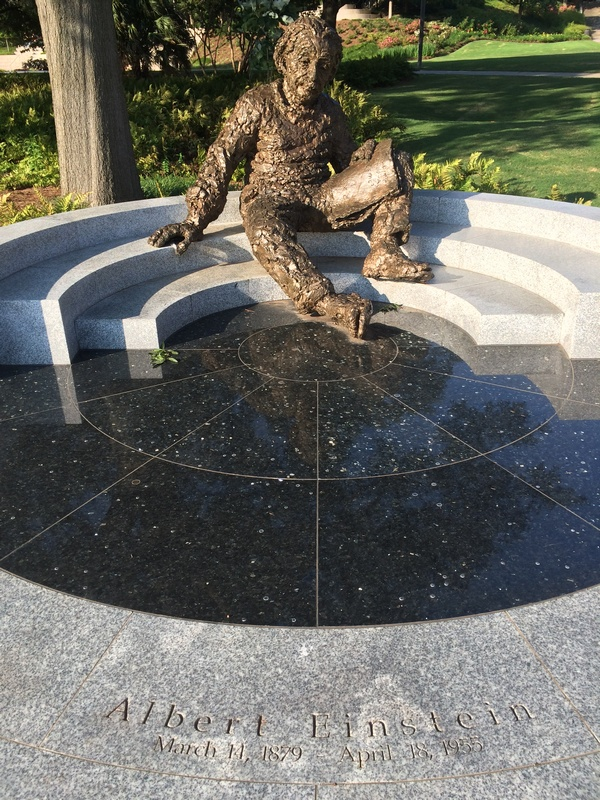Written" on the papers Einstein is holding: =================================== Rμν - (1/2)gμνR = κTμν (the theory of GR) eV = hν - A (the PhotoElectric Effect) E = mc2 (the Equivalence of Energy and Matter) =================================== Correlates to: ========== GR QM SR ==========
Einstein's genius changed science's perception of gravity: General relativity has grown more important than it was in Einstein's day (Oct 2015),
Einstein and Quantum Mechanics:  It’s Not What You Think- A.D.S.
===
Einstein is well known for his rejection of quantum mechanics in the form it emerged from the work of Heisenberg, Born and Schrodinger in 1926. Much less appreciated are the many seminal contributions he made to quantum theory prior to his final scientific verdict, that the theory was at best incomplete. Einstein fathered many conceptual breakthroughs and they need to be placed in historical context. Stone argues that Einstein, much more than Planck, introduced the concept of quantization of energy in atomic mechanics. Einstein proposed the photon, the first force-carrying particle discovered for a fundamental interaction, and put forward the notion of wave-particle duality, based on sound statistical arguments 14 years before De Broglie’s work. He was the first to recognize the intrinsic randomness in atomic processes, and introduced the notion of transition probabilities, embodied in the A and B coefficients for atomic emission and absorption. He also preceded Born in suggesting the interpretation of wave fields as probability densities for particles, photons, in the case of the electromagnetic field. Finally, stimulated by Bose, he introduced the notion of indistinguishable particles in the quantum sense and derived the condensed phase of bosons, which is one of the fundamental states of matter at low temperatures. His work on quantum statistics in turn directly stimulated Schrodinger towards his discovery of the wave equation of quantum mechanics. It was only due to his rejection of the final theory that he is not generally recognized as the most central figure in this historic achievement of human civilization - quantum mechanics.
===
An Einstein We Didn't Know: Despite his famed line about God ‘not playing dice,’ evidence in letters shows that Einstein was the living spirit behind quantum mechanics (May 2018),

Now, some basics about SR 4-Vectors...
4-Vector Vμ = (V0,Vi) = (V0,V1,V2,V3)

 V0 V1 V2 V3 temporal part = V0 spatial part = Vi

#### SR Light Cone

| time-like interval(+)( Vμν )

|            / light-like interval(0 = null)( Nμν )
worldline
.......|....... c       --- space-like interval(-)( Hμν )
\..future./
\....|..../
\..|../
\|/now,here( ημν )
/|\
/..|..\    elsewhere
/....|....\
/...past...\
.......|...... -c

with:
( Vμν ) as the "Vertical" = Temporal Projection Tensor
( Hμν ) as the "Horizontal" = Spatial Projection Tensor
( Nμν ) as the "Null" = Lightlike Projection Tensor
( ημν ) as the Minkowski Metric

#### SR 4-Vectors - Conventions and Properties

*Note* Numeric subscripts and superscripts on variables inside the vector parentheses typically represent tensor indices, not exponents
In the following, I use the Time-0th-Positive SR metric sign convention ημν =   ημν =   DiagnolMatrix[+1,-1,-1,-1]
I use this primarily because it reduces the number of minus signs in Lorentz Scalar Magnitudes, since there seem to be more time-like physical 4-Vectors than space-like.
Also, this sign convention is the one matched by the QM Schrödinger Relations later on...

I always choose to have the 4-Vector refer to the upper index tensor of the same name. {eg.A = Aμ}
In addition, I like the convention of having the (c) factor in the temporal part for correct dimensional units. {eg. 4-Position R = (ct,r)}
This allows the SR 4-Vector name to match the classical 3-vector name, which is useful when considering Newtonian limiting cases.
I will use UPPER case bold for 4-Vectors, and lower case bold for 3-vectors.

All SR 4-Vectors have the following properties:
==================================

A = Aμ = (a0,ai) = (a0, a) = (a0,a1,a2,a3)     → (at,ax,ay,az): A typical 4-Vector

Aμ = (a0,ai) = (a0,-a) = (a0 ,a1, a2, a3)  → (at, ax, ay, az)
= (a0,-a) = (a0,-a1,-a2,-a3) → (at,-ax,-ay,-az): A typical 4-covector

where Aμ = ημνAν  and  Aμ = ημνAν: Tensor index lowering and raising with the Minkowski Metric

A·B = Aμ ημν Bν = Aν Bν = Aμ Bμ = +a0b0-a·b = +a0b0-a1b1-a2b2-a3b3 The Scalar Product relation, used to make Invariant Lorentz Scalars
If the scalar product is between tensors with multiple indices, then one should use tensor indices for clarity, otherwise the equation remains ambiguous.
{eg. U·Fμν = Uα·Fμν = ? → UαηαμFμν =   UμFμν  or  UαηανFμν =   UνFμν }
Importantly, A·B = (a0ob0o) = Ao·Bo and  A·A = (a0o)2 = Ao·Ao, the Lorentz Scalar Product can quite often be set to the "rest values" of the temporal component.
This occurs when the 4-Vector A is Lorentz-Boosted to a frame in which the spatial component is zero:  A = (a0, a) → Ao = (a0o, 0)
[A·B > 0] → Time-Like
[A·B = 0] → Light-Like / Photonic / Null
[A·B < 0] → Space-Like

The Invariant Rest Value of the Temporal Component Rule:
==========================================
β = v/c = u/c
4-UnitTemporal T = γ(1,β) = U/c
4-Velocity U = γ(c,u) = cT
Generic 4-Vector A = (a0,a)
A·T = (a0, a)·γ(1,β) = γ(a0*1 - a·β) = γ(a0 - a·β) = (1)(a0o - a·0) = a0o
A·T = a0o
The Lorentz Scalar product of any 4-Vector with the 4-UnitTemporal gives the Invariant Rest Value of the Temporal Component.
This makes sense from a vector viewpoint - you are taking the projection of the generic vector along a unit-length vector in the time direction.
A·U = c*a0o
The Lorentz Scalar product of any 4-Vector with the 4-Velocity gives c*Invariant Rest Value of the Temporal Component.
It's the same thing, just multiplied by (c).
I will call these ( A·T = a0o or A·U = c*a0o ) the "Invariant Rest Value of the Temporal Component Rule".
This will get used extensively later on...
There is an analogous relation with the 4-UnitSpatial.

=================================
4-Position R = (ct,r)
Generic 4-Vector A = (a0,a), which is not a function of R

A·R = (a0,a)·(ct,r) = (a0*ct - a·r) = Θ is equivalent to [Θ] = [A·R] = A

{ A·R = Θ } <→ { [Θ] = A }

Proof:
Let A·R = Θ
[Θ] = [A·R] = [A]·R + [R] = (0) + Aκ·∂μ[Rν] = Aκ·ημν = Aκηκμημν = Aμημν = Aν = A

Let [Θ] = A
A·R = (a0,a)·(ct,r) = (a0*ct - a·r) = (t[Θ]/c*ct + [Θ]·r) = (t[Θ]*t + [Θ]·r) = Θ

*Note*
f = f(t,x)  →  df = (∂tf) dt + (∂xf) dx
f = ∫df = ∫(∂tf) dt + ∫(∂xf) dx
f → (∂tf) ∫dt + (∂xf) ∫dx = ∂tf *t + ∂xf *x   {if the partials are constants wrt. t and x, which was the condition from A not a function of R}

This comes up in the SR Phase and SR Analytic Mechanics.

Basis Representation & Independence (Manifest Covariance):
===========================================
When the components of the 4-Vector { A }are in (time scalar,space 3-vector) form { (a0,a) }then the 4-Vector is in spatial basis invariant form.
Once you specify the spatial components individually, you have picked a basis or representation.  I indicate this by using { → }.
e.g. 4-Position X = (ct,x) = {Space Basis independent representation}
→ (ct,x,y,z) = {Cartesian/rectangular representation}
→ (ct,r,θ,z) = {Cylindrical representation}
→ (ct,r,θ,φ) = {Spherical representation}
These can all indicate the same 4-Vector, but the components of the 4-Vector will vary in the different bases.

Now, once you are in a space basis invariant form, e.g. X = (ct,x), you can still do a Lorentz boost and still have the same 4-Vector X.
It is only when using 4-Vectors directly, (eg. X·Y, X+Y), that you have full Spacetime Basis Independence.
Knowing this, we try to find as many relations as possible in 4-Vector and Tensor format, as these are applicable to all observers.
=================================

Since the language of SR is beautifully expressed using 4-Vectors, I will use that formalism. There are quite a few different variations of 4-Vectorsthat can correctly describe SR. I use the one that has only real (non-complex) notation throughout SR. The imaginary unit ( i ) is introduced only at the last step, which gives QM. As you can note from the outline,there are only a few steps necessary. By the way, SR is an excellent approximation for the majority of the currently known universe, including on the surface of Earth.  It is only in the regions of extreme curvature,such as near a stellar surface or black hole, that GR is required.

See the 4-Vectors Reference for more reasoning on the choice of notation, and for more on four-vectors in general.

#### Interesting points include:

*All events, at which there may or may not be particles, massive or massless, have a 4-velocity magnitude of c, the speed of light.
*A number of particle properties are simply constants times another property.
*Wave-particle duality occurs purely within SR - ex. Relativistic Optics,Relativistic Doppler Effect.
*Fields occur purely due to SR Potential Momentum.
*QM is generated simply by allowing the particles to have imaginary/complex components within spacetime.
*The Quantum Superposition Principle, usually assumed as axiomatic, is a consequence of the Klein-Gordon equation being a linear wave PDE.

#### Notation and Properties of SR 4-Vectors

gμν = gμν → ημν =  DiagnolMatrix[1,-1,-1,-1] Minkowski Spacetime Metric: This is the "flat"spacetime of SR

All SR 4-Vectors have the following properties:
A = Aμ = (at,ax,ay,az) = (a0,a1,a2,a3) = (a0,a) A typical 4-Vector
Aμ = (at,ax,ay,az) = (a0,a1,a2,a3) = (at,-ax,-ay,-az) = (a0,-a1,-a2,-a3) = (a0,-a) A typical 4-covector; we can always get the 4-Vector form with Aμ = ημνAν
A·B = ηuv Au Bν = AνBν = Aμ Bμ = +a0b0-a1b1-a2b2-a3b3 = +a0b0-a·b :The Scalar Product relation,used to make Invariant Lorentz Scalars
[A·B > 0] → Time-Like
[A·B = 0] → Light-Like / Null
[A·B < 0] → Space-Like

#### Useful Quantities

γ[v] = 1 / √[1-(v/c)2] : Lorentz Scaling Factor (gamma factor)
τ[v,t] = t / γ : Proper Time
Sqrt[1+x] = √[1+x] ~ (1+x/2) for |x|<<1 : Math relation often used to simplify Relativistic eqns. to Newtonian eqns.

#### Fundamental/Universal Physical Constants (Lorentz Scalars)

c = Speed of Light
ћ = h/2π = Planck's Reduced Const aka. Dirac's Const
mo = Particle Rest Mass (varies with particle type)
q = Particle Charge (varies with particle type)

#### Fundamental/Universal Physical 4-Vectors (Lorentz Vectors)

(this notation always places the c-factor in the time-like part, and the name goes with the space-like part)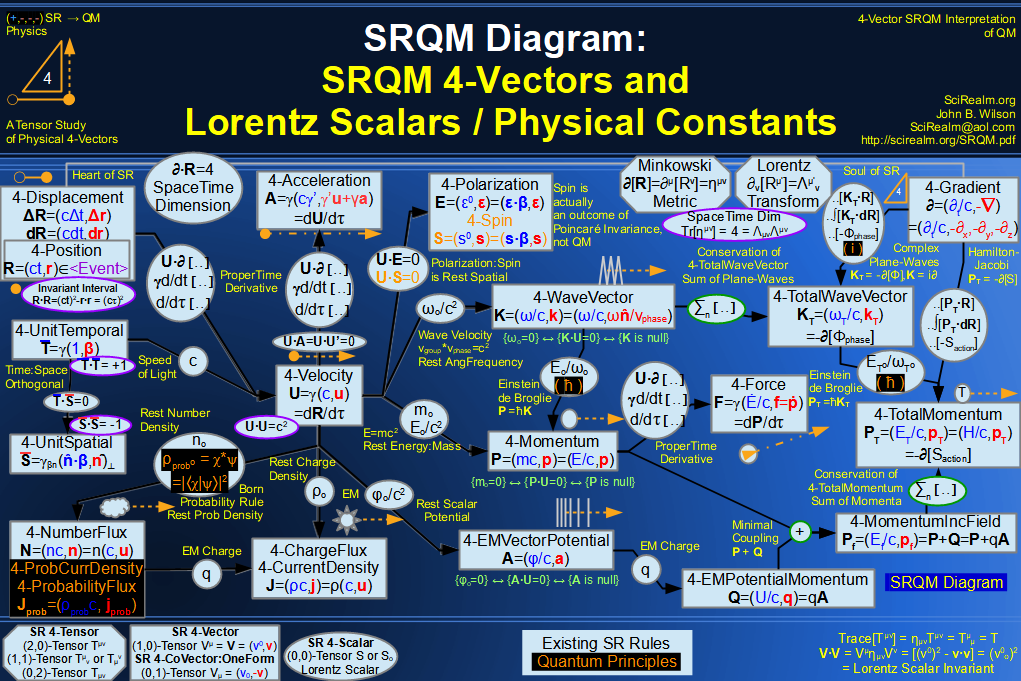4-Position R = (ct,r)
4-Velocity U = γ(c,u)
4-Momentum P = (E/c,p) = (mc,p) = γmo(c,u)
4-CurrentDensity J = (cρ,j) = γρo(c,u)
4-WaveVector K = (ω/c,k) = (ω/c,nω/vphase) = (ω/c)(1,β) = (1/cT,n/λ)
4-Gradient = (t/c,-) → (/ct,-/x,-/y,-/z) = (t/c,-x,-y,-z)
4-VectorPotential A = (φ/c,a)
4-PotentialMomentum Q = qA = q(φ/c,a) = (U/c,q) *includes effect of charge q*
4-TotalMomentum PT = (H/c,pT) = P +Q = P + qA
4-TotalGradient D = + iq/ћA = 4-Gradient + effects of Vector Potential

#### Fundamental/Universal Relations

R = R
U = dR/dτ "4-Velocity is the derivative of 4-Position wrt. proper time"
P = moU
J = ρoU
K = P / ћ
= -iK
Q = qA
PT = (H/c,pT) = P +Q
D = + iq/ћA "whereA is the (EM) vector potential and q is the (EM) charge"

#### Derived Physical Constants (Scalar Products of Lorentz Vectors give Lorentz Scalars)

R·R = (Δs)2 = (ct)2-r·r = (ct)2-|r|2
U·U = (c)2
P·P = (moc)2 : J·J = (ρoc)2
K·K = (moc/ћ)2
· = (-imoc/ћ)2 = -(moc/ћ)2Now then, how do we get QM out of SR?

Start with a special relativistic spacetime for which the invariant measurement interval is given byR·R = (Δs)2 = (ct)2-r·r = (ct)2-|r|2.
This is just a "flat" Euclidean 3-space with an extra, reversed-sign dimension, time, added to it.
This interval is Lorentz Invariant.
In this convention, space-like intervals are (-)negative, time-like intervals are (+)positive, and light-like intervals are (0)null.
One can say that the universe is the set of all possible events in spacetime.

#### Events/Particles:

All of the Special Relativistic notation applies to the concept of events.
Events are simply points in spacetime.  The measurement interval between points is an invariant.
Now, let's examine the interesting events...
There exist particles (which can carry information) that move about in this spacetime.
Each particle is located at an event (a time and a place) 4-Position R = (ct,r).
The factor of (c) is inserted in the time part to give the correct,consistent dimension of length to this 4-Vector.
In fact, every SR 4-Vector has this constant c-factor to give consistent dimensions.
A particle is simply a self-sustaining event, or more correctly a worldline of connected events, which "carries" information forward in time.
The information that a particle can "carry" include mass, charge, any of the various hypercharges, spin, polarization, phase, frequency, energy, etc..
These are the particles' properties.

#### Motion/Dynamics:

Let these particles be able to move around within the spacetime.
The 4-Velocity of an event is given by U = dR/dτ, or the total derivative of the 4-Position with respect to its Proper Time.
This gives the 4-Velocity U = γ(c,u), with γ(v) = 1 / √[1-(v/c)2].
This particle, if its rest mass mo > 0, moves only in the direction of +time along its own worldline Uworldline = (c,0).
Interestingly, all stationary (v = 0) massive particles move into the future at c, the Speed of Light;
If the particle has rest mass mo = 0, it moves in a null or light-like direction. This is neither along time nor along space, but"between" them.
These light-like particles, with a v = c, have a 4-Velocity: Ulight-like = Infinite c(1,), where is a unit space vector.
Since this is rather undefined, we will use the 4-Wave Vector, introduced later, to describe photons.
A particle only has a spatial velocity u with respect to another particle or an observer.
We have the relation √(U·U) = c. This says that the magnitude of the 4-velocity is c, the speed of light. This result is general, massive or massless!
What all this means is that all light-like particles live on the "surface"null-space of the Light Cone, between time and space,
while all massive particles live within the "interior" the Light Cone.

#### SR Light Cone

| time-like interval(+)( Vμν )

|            / light-like interval(0 = null)( Nμν )
worldline
.......|....... c       --- space-like interval(-)( Hμν )
\..future./
\....|..../
\..|../
\|/now,here( ημν )
/|\
/..|..\    elsewhere
/....|....\
/...past...\
.......|...... -c

#### Mass/Energy/Momentum:

One of the basic properties of particles is that of mass. Each particle has a rest mass mo.
Rest mass is simply the mass as measured in a frame at rest with respect to an observer.
This mass, along with the velocity of a particle, gives 4-Momentum P = moU.
Nature seems to indicate that one of the fundamental conservation laws is the Conservation of 4-Momentum.
This comes from the idea that a system remains invariant under time or space translations in an isotropic, homogeneous universe.
The sum of all particle 4-Momenta involved in a given interaction is constant; it has the same value before and after a given interaction.
The 4-Momentum relation P = moU gives 4-Momentum P = (E/c,p) = moU = γmo(c,u).
This gives the Einstein Mass-Energy relation, E = γmoc2, or E = mc2 where m = (γmo).
Note that for light-like particles, the result using this formula is undefined since Elight-like = Infinite * 0 * c2.
Presumably, the m = (γmo) factor must scale in some way (i.e. like a delta function) to give reasonable results.
Also, there is a lot of confusion over whether (m) is the actual mass or not.
A simple thought experiment clears this up.  Imagine an atom at rest, having rest mass mo.
Now imagine an observer moving past the atom at near light speed.
The apparent mass of the atom to the moving observer is m = (γmo).
Now imagine this observer accelerating to ever greater speeds.
The atom is sitting happy and unchanging in its own rest frame.
However, once the observer is going fast enough, this apparent mass m = (γmo) could be made to exceed that necessary to create a black hole.
As that would be an irreversible event, the gamma factor (γ) must simply be a measure of the relative velocities of the two events.
So, the true measure of actual mass is just the rest mass (mo).

#### Waves/Null-Particles:

The energy of null/light-like particles can be obtained another way.
It turns out that every photon (light particle) has associated with it a 4-WaveVector K = (ω/c,k), with ω = temporal angular frequency.
Through the efforts of Planck, Einstein, and de Broglie, it was discovered that K = P / ћ = (ω/c,k) = 1/ћ (E/c,p).
We should note here that (h) (also ћ = h/2π) is an empirical constant, which can be measured with no assumptions about QM, just as (c) is an empirical constant which can be measured with no assumptions about SR.
Planck discovered (h) based on statistical-mechanics/thermodynamic considerations of the black-body problem.
Einstein applied Planck's idea to photons in the photoelectric effect to give E = ћ ω and the idea of photons as particle quanta.
de Broglie realized that every particle, massive or massless, has 3-vector momentum p = ћk.
Putting it all together naturally produces 4-Vector P = ћK = (E/c,p) = ћ(ω/c,k).
Note also that the 4-WaveVector (a wave-like object) is just a constant, (ћ), times the 4-Momentum (a particle-like object).
This means that photons, or other massless quanta, can act like localized particles and massive quanta can act like non-localized waves.
That gives the Mass-Energy relation for all kinds of particles, ( E = γmoc2 = ћ ω ), and also gives the relation for m = (γmo) = ω ћ/c2 = (γωo)ћ/c2.
Note that massive particle would have rest frequency ωo, which would look like (γωo) to an observer, while massless particles simply have frequency (ω).
This leads into the wave-particle duality aspect of nature, and we haven't even gotten to QM yet!

Note: "There is a duality of particle and wave even in classical mechanics, but the particle is the senior partner, and the wave aspect has no opportunity to display its unique characteristics."
- Goldstein, Classical Mechanics 2nd Ed., pg 489
(The relation between geometrical optics and wave mechanics using the Hamilton-Jacobi Theory).

I need to emphasize here that the 4-WaveVector can exist as an entirely SR object (non-QM). It can be derived in terms of periodic motion, where families of surfaces move through space as time increases, or alternately,as families of hypersurfaces in spacetime, formed by all events passed by the wave surface. The 4-WaveVector is everywhere in the direction of propagation of the wave surfaces. From this structure, one obtains relativistic/wave optics, without ever mentioning QM.

I believe that there is more to the 4-WaveVector than other people have figured on (i.e. more importance to the overall phase Φ of the waves). More on that later...
Also, the question always arises: What is waving? I assume that it is simply an internal property of a particle that happens to be cyclic. This would allow all particles to be "waves", or more precisely to have a cyclic period, without the need for a medium to be waving in. Also, note that the phase of the 4-WaveVector was not defined. Presumably, (2π) of 4-WaveVec's could have the same 4-Vector K .  However, another interpretation could be the symmetry between 4-Vectors and One-Forms, where the 4-Vectors consist of "arrows" and one-forms consist of "parallel lines".  The length of arrow along the lines is the dot-product operation, which results in a Lorentz scalar number.
Also, it is at this step that I believe a probabilistic description is being imposed on the physics.

#### Spacetime Structure:

Now, let's get to the really tough stuff.
There is a thing called the 4-Gradient = μ = (t/c,-) = (t/c,-del) → (/ct,-/x,-/y,-/z) = (t/c,-x,-y,-z) , where ∂ is the partial derivative function.
It tells you about the changes/variations in the "surface" of spacetime.
This 4-Vector is significantly different from the others. It is a function that acts on a value, not a value itself.
It also has a negative sign in the space component, for the upper tensor index, unlike the other "physical type" vectors.

·X = /ct[ct]+∇·x = t/t+∇·x = 4.
This tells us the number of spacetime dimensions.

When it is applied to the 4-CurrentDensity, it leads to the Conservation of Charge equation.
·J = /ct[cρ]+·j = ρ/t+·j = 0.
This says that the change in charge-density with respect to time is balanced by the divergence or spatial flow of current-density.

The same thing can be applied to particle 4-Momentum of a particle:
·P = /ct[E/c]+·p = (1/c2)E/t +·p = 0.
E/t+c2·p = 0.
This says that the change in energy with respect to time is balanced by the divergence or spatial flow of momentum.
In fact, this is the 4-Vector Conservation of Momentum Law.
Energy is neither created nor destroyed, only transported from place to place in the form of momentum.
This is the strong, local form, of conservation - the continuity equation.

Additionally, U·∂ = γ(∂/∂t +) = γ d/dt = d/dτ
Showing that the derivative w.r.t. Proper Time is a Lorentz Scalar Invariant.

The 4-Gradient = (t/c,-) = (t/c,-del) is an SR functional that gives the structure of Minkowski Spacetime.
The Lorentz Scalar Product· = (t/c,-)·(t/c,-) = (t/c)2 -·
gives the d'Alembertian equation / wave equation.  The d'Alembert operator is the Laplace operator of Minkowski Space.
Despite being a functional, the d'Alembertian is still a Lorentz Scalar Invariant.
The Green's function G[X-X'] for the d'Alembertian is defined as (·)G[X-X'] = δ4[X-X']

So, given all the above, we have clearly shown that is SR 4-Vector, not something from QM.

Now, let's perform some pure SR math based on our SR 4-Vector knowledge

4-Gradient = (∂t/c,-)                       ∂∙∂ = (∂t/c)2∙∇
4-PositionX = (ct,x)                              X∙X = ((ct)2 -x∙x)
4-VelocityU = γ(c,u)                             U∙U = γ2(c2 -u∙u) = (c)2
4-MomentumP = (E/c,p) = (Eo/c2)U      P∙P = (E/c)2 -p∙p = (Eo/c)2
4-WaveVectorK = (ω/c,k) = (ωo/c2)U    K∙K = (ω/c)2 -k∙k = (ωo/c)2

∂∙X = (∂t/c,-)∙(ct,x) = (∂t/c[ct]-(-∇∙x)) = 1-(-3) = 4
U∙∂ = γ(c,u)∙(∂t/c,-) = γ(∂t+u∙∇) = γ(d/dt) = d/dτ
[X] = (∂t/c,-)(ct,x) = (∂t/c[ct],-[x]) = Diag[1,-1] = ημν
[K] = (∂t/c,-)(ω/c,k) = (∂t/c[ω/c],-[k]) = Diag[0,0] = []
K∙X = (ω/c,k)∙(ct,x) = (ωt –k∙x) = Φ
[K∙X] = [K]∙X+K∙∂[X] = K = [Φ]
(∂∙∂)[K∙X] = ((∂t/c)2∙∇)(ωt–k∙x) = 0
(∂∙∂)[K∙X] = ∂∙([K∙X]) = ∂∙K = 0

Now, let's make a SR function f
let f = aeb(K∙X), which is just a simple exponential function of 4-Vectors
then [f] = (bK)aeb(K∙X) = (bK)f
and ∂∙∂[f] = b2(K∙K)f = (bωo/c)2f
Note that { b = -i } is an interesting choice – it leads to SR Plane Waves,which we observe empirically, e.g. EM Plane Waves...
This gives:
[f] = (-iK)ae-i(K∙X) = (-iK)f
[f] = (-iK)f
= -iK

#### Now comes Quantum Mechanics (QM)!

Now then, based on empirical evidence:
QM (and enhancements like QED and QFT) have given the correct calculation/approximation of more phenomena than any other theory, ever.
We have the following simple relation: = -iK or K = i.
This innocent-looking, very simple relation gives all of Standard QM.
It does this in a number of ways, one of which is by providing the Schrödinger relation P = ћK = i ћ.
In component form this is (E = i ћ /t) and (p = -iћ).
These are the standard operators used in the Schrödinger/Klein-Gordon eqns (as well as other relativistic quantum field equations), which are the basic QM description of physical phenomena.
This essentially gives the Operator Formalism, Unitary Evolution, and Wave Structure Axioms of QM, which governs how the state of a quantum system evolves in time.
We have:
[] = -iK: Operator Formalism
= [ -i ]K: Unitary Evolution
= -i [K]:Wave Structure

One also finds that SR events oscillate with a rest freq that is proportional to rest mass.
U·∂ = γ(∂/∂t +u·∇) = γ d/dt = d/dτ
d/dτ = U·∂
d/dτ = (-iK)
d/dτ = (-i/ћP)
d/dτ = (-imo/ћ U)
d/dτ = (-imo/ћ )U·U
d/dτ = (-imoc2/ћ)
d/dτ = (-iωomoc2/ћωo)
d/dτ = (-iωo)
d2/dτ2 = -(ωo)2
Now, apply this to the 4-Position...
d2X/dτ2 = -(ωo)2X
This is the differential equation of a relativistic harmonic oscillator!
Quantum events oscillate at their rest-frequency.
Likewise for the momenta:
d2P/dτ2 = -(ωo)2P

Next, let's look at Quantum Commutation Relations...
4-Position X = (ct,x)
Then, purely from math...
==================
Let ψ be an arbitrary function.
X
[ψ] = Xψ,
[ψ] = [ψ]
X[[ψ]] = X∂[ψ]
[X[ψ]] = [Xψ] = [X]ψ +X∂[ψ]
[Xψ]-X∂[ψ] = [X
now with commutator notation
[,X]ψ = [X
And since ψ was an arbitrary function...
[,X] = [X]

[,X] = [X] = (∂t/c,-)[(ct,r)] = (∂t/c,-∂x,-∂y,-∂z)[(ct,x,y,z)] = Diag[1,-1,-1,-1] = ημν = Minkowski Metric
[,X] = ημν = Minkowski Metric

==================
At this point,we have established purely mathematically, that there is a non-zero commutation relation between the SR 4-Gradient and SR 4-Position.
Then, from our empirical measurements...
we find that ∂ = - iK so

[,X] = ημν[-iK,X] = ημν
- i[K,X] = ημν
[K,X] = iημν

Then, from our empirical measurements...we know that K = (1/ћ)P
[K,X] = iημν[(1/ћ)P,X] = iημν
(1/ћ)[P,X] = iημν
[P,X] = iћημν

[Xμ,Pν] = - iћημν

and, looking at just the spatial part

[xj,pk] = iћδjk

Hence, we have derived the standard QM commutator rather than assume it as an axiom...

Let's summarize a bit:
We used the following relations:(particle/location→movement/velocity→mass/momentum→wave duality→spacetime structure)
With the exception of 4-Velocity being the derivative of 4-Position, all of these relations are just constants times other 4-Vectors.
 R = (ct,r) particle/location U = dR/dτ movement/velocity P = moU mass/momentum K = 1/ћP wave duality ∂ = -iK spacetime structure

By applying the Scalar Product law to these relations, we get:
U·U = (c)2
P·P = (moc)2
K·K = (moc/ћ)2
· = (-imoc/ћ)2 = -(moc/ћ)2

Let's look at that last equation.
· = (/ct,-)·(/ct,-) = 2/c2t2-· = -(moc/ћ)2,
 ∂2/c2∂t2 = ∇·∇-(moc/ћ)2

This is the basic, free-particle, Klein-Gordon equation, the relativistic cousin of the Schrödinger equation!
It is the relativistically-correct, quantum wave-equation for spinless (spin 0) particles.
We have apparently discovered QM by multiplying with the imaginary unit, ( i ).
Essentially, it seems that allowing SR relativistic particles to move in an imaginary/complex space is what gives QM.
At this point, you have the simplest relativistic quantum wave equation.
The principle of quantum superposition follows from this, as this wave equation (a linear PDE) obeys the superposition principle.
The quantum superposition axiom tells what are the allowable (possible) states of a given quantum system.
I believe that the only other necessary postulate to really get all of standard QM is the probability interpretation of the wave function,
and that likely is simply reinterpretation of the continuity equation:
·J = /ct(cp) +·j = p/t +·j = 0, where J is taken to be a "particle"current density.

The Klein-Gordon equation is more general than the Schrödinger equation,but simplifies to the Schrödinger equation in the (|v| << c) limit.
Also, extensions into EM fields (or other types of relativistic potentials) can be made using D = + iq/ћA with A as the 4-EMVectorPotential and q is the EM charge,
and allowingD·D = -(moc/ћ)2 to be the more correct EM quantum wave equation.

Now, let's back up a bit to P·P = (moc)2
P·P - (moc)2 = 0
(E/c)2 -p·p - (moc)2 = 0
E2 - c2p·p - (moc2)2 = 0
this can be factored into...
[ E - cα·p - β(moc2) ] [ E + cα·p+ β(moc2) ] = 0
where:
E and p are quantum operators,
α and β are matrices which must obey αiβ = -βαiiαj = -αjαi, αi2 = β2 = I
The left hand term can be set to 0 by itself, giving...
[ E - cα·p - β(moc2) ] = 0, which is the Dirac equation, which is correct for spin 1/2 particles

#### Potentials/Fields:

Let's back up to the 4-Momentum equation.  Momentum is not just a property of individual particles, but also of fields.
These fields can be described by 4-Vectors as well.
One such relativistically invariant field is the 4-VectorPotential A, which is itself a function of 4-Position X.
Typically, we deal with the  Electromagnetic (EM) 4-VectorPotential, but it could be any kind of relativistic charge potential...
4-VectorPotential A[X] = A[(ct,x)] = (φ/c,a) = (φ[(ct,x)]/c, a[(ct,x)]), where the [(ct,x)] means is a function of time t and position x.
While a particle exists as a worldline over spacetime, the 4-VectorPotential exists over all spacetime.
The 4-VectorPotential can carry energy and momentum, and interact with particles via their charge q.

#### PotentialMomentum:

One may obtain the PotentialMomentum 4-Vector by multiplying by a charge q,Q = qA
4-PotentialMomentum Q = qA = q(φ/c,a) = (U/c,p)
The 4-TotalMomentum is then given by PT = P +Q
This includes the momentum of particle and field, and it is the locally conserved quantity.
4-TotalMomentum PT = (H/c,pT), where these are the TotalEnergy = Hamiltonian and 3-TotalMomentum.
P = PT -Q = moU
Now working back, we can make our dynamic 4-Momentum more generally,including the effects of potentials.
4-Momentum P = (E/c,p) = (H/c - U/c,pT-pEM) = (H/c - qφ/c,pT - qa)
The dynamic 4-momentum of a particle thus now has a component due to the 4-VectorPotential,
and reverts back to the usual definition of 4-momentum in the case of zero 4-VectorPotential.
Likewise, following the same path as before...
K = P / ћ
4-WaveVector K = (ωT/c -(q/ћ)φ/c,kT - (q/ћ)a)
= -iK
4-Gradient = (T/ct- (iq/ћ)φ/c,-T - (iq/ћ)a) = (t/c,-)
This is the concept of "Minimal Coupling"
Minimal Coupling can be extended all the way to non-Abelian gauge theories,
and can be used to write down all the interactions of the Standard Model of elementary particles physics between spin-1/2"matter particles" and spin-1 "force particles"
Minimal Coupling applied to the Dirac Eqn. leads to the Spin Magnetic Moment-External Magnetic Field coupling W = -γeS·B, where γe = qe/me,the gyromagnetic ratio.
The corrections to the anomalous magnetic moment come from minimal coupling applied to QED

In addition, we can go back to the velocity formula:

u = c2 (p)/(E) = c2 (pT - qa)/(H - qφ)

#### Lagrangian/Hamiltonian Formalisms:

see Relativistic Lagrangian Mechanics
Relativistic Beta Factor β = u/c
Relativistic Gamma Factor γ = 1/√[1-β2]

The whole Lagrangian/Hamiltonian connection is given by the relativistic identity:
γ = 1/√[1 - β2]
γ2 = 1/(1 - β2)
(1 - β22 = 1
2 - γ2β2) = 1
2 - 1) = γ2β2
( γ - 1/γ ) = (γβ2)
Now multiply by your favorite Lorentz Scalars... In this case for a free relativistic particle...
( γ - 1/γ )(P·U) = ( γβ2)(P·U)
( γ - 1/γ )(moc2) = ( γβ2)(moc2)
( γmoc2 - moc2/γ ) = γmoc2β2
( γmoc2 - moc2/γ ) = γmou2
( γmoc2) + (- moc2/γ ) = γmou·u
( γmoc2) + (- moc2/γ ) = (p·u)
( H ) + ( L )  = (p·u)
The Hamiltonian/Lagrangian connection falls right out.
Note that neither (H) nor (L) are scalar invariants, due to the extra (γ) factors.

Now, including the effects of a potential:
4-VectorPotential A = (φ/c,a) { = (φEM/c,aEM) for EM potential }
4-PotentialMomentum Q = qA due to 4-VectorPotential acting on charge (q)
4-TotalMomentum of System PT = P + Q = P + qA = moU + qA = (H/c,pT) = (γmoc + qφ/c,γmou + qa)
A·U = γ(φ - a·u) = φo P·U = γ(E - p·u) = Eo PT·U = Eo + qφo = moc2 + qφo
I assume the following as a valid SR relation:
A = (φo/c2)U = (φ/c,a) = (φo/c2)γ(c,u) = ((γφo/c), (γφo/c2)u) giving (φ = γφo and a = (γφo/c2)u)
This is analogous to P = (Eo/c2)U
( γ - 1/γ )(PT·U) = ( γβ2)(PT·U)
γ(PT·U) + -(PT·U)/γ  = ( γβ2)(PT·U)
γ(PT·U) + -(PT·U)/γ  = (pT·u)
( H ) + ( L ) = ( pT·u )
 Relativistic Hamiltonian H = γ(PT·U) Relativistic Lagrangain L = -(PT·U)/γ pT·u = ( γβ2)(PT·U) = H + L = γ(PT·U) + -(PT·U)/γ H = γ(PT·U) H = γ((P + Q)·U) H = γ(P·U + Q·U) H = γP·U + γQ·U H = γmoU·U + γqA·U H = γmoc2 + qγφo H = γmoc2 + qφ H = ( γβ2 + 1/γ )moc2 + qφ H = ( γmoβ2c2 + moc2/γ) + qφ H = ( γmou2 + moc2/γ) + qφ H = p·u + moc2/γ  + qφ H = E + qφ H = ±c√[mo2c2 + p2] + qφ H = ±c√[mo2c2 + (pT -qa)2] + qφ H = ±moc2√[1 + (pT -qa)2/(mo2c2)] + qφ L = -(PT·U)/γ L = -((P + Q)·U)/γ L = -(P·U + Q·U)/γ L = - P·U/γ - Q·U/γ L = -moU·U/γ - qA·U/γ L = -moc2/γ - qA·U/γ L = -moc2/γ - q(φ/c,a)·γ(c,u)/γ L = -moc2/γ - q(φ/c,a)·(c,u) L = -moc2/γ - q(φ - a·u) L = -moc2/γ - qφ + qa·u L = -moc2/γ - qφo/γ L = -(moc2 + qφo)/γ H + L = γ(PT·U) - (PT·U)/γ H + L = (γ - 1/γ)(PT·U) H + L = ( γβ2)(PT·U) H + L = ( γβ2)((P + Q)·U) H + L = ( γβ2)(P·U + Q·U) H + L = ( γβ2)(moc2 + qφo) H + L = (γmoβ2c2 + qγφoβ2) H + L = (γmou·uc2/c2 + qφoγu·u/c2) H + L = (γmou·u + qa·u) H + L = (p·u + qa·u) H + L = pT·u Rest Hamiltonian Ho = (PT·U) = H/γ Rest Lagrangain Lo = -(PT·U) = γL Ho + Lo = 0

The non-relativistic Hamiltonian Hnon-rel is an approximation of the relativistic Hamiltonian H:
H = γ(moc2 + qφo)
H = (1/√[1-(v/c)2])(moc2 + qΦo) ~ [1 + (v/c)2/2])(moc2 + qφoEM) = (moc2 + qφo) + (1/2)(moc2v2/c2 + qφov2/c2)  ~ (moc2 + qφo) + (1/2)(mov2 + 0)
H ~ (1/2)(mov2) + (moc2 + qφo)
H ~ (Kinetic) + (Rest + Potential) = T + V {for |v| << c}
Hnon-rel = T + V

The non-relativistic Lagrangian Lnon-rel is an approximation of the relativistic Lagrangian L:
L = -(moc2 + qφo)/γ
-L = (moc2 + qφo)/γ = √[1-(v/c)2](moc2 + qφo) ~ (moc2 + qφo) - (1/2)(moc2v2/c2 + qφov2/c2)  ~ (moc2 + qφo) - (1/2)(mov2 + ~0 )
L ~ (1/2)(mov2) - (moc2 + qφo)
L ~ (Kinetic) - (Rest + Potential) = T - V {for |v| << c}
Lnon-rel = T - V

Thus, (H ~ T + V = Hnon-rel ) and (L ~ T - V = Lnon-rel ) only in the non-relativistic limit (|v| << c)
H + L ~ (T + V) + (T - V) = 2T = 2 (1/2 mou·u) = p·u
Thus, ( H ) + ( L ) = (p·u) is always true, in both the relativistic and non-relativistic case.

H = ± moc2√[1 + (pT -qa)2/(mo2c2)] + qφ
H ~ ± moc2[1 + (pT -qa)2/(2mo2c2)] + qφ for |(pT -qa)2/(moc)2| << 1
H ~ ± [moc2 + (pT -qa)2/(2mo)] + qφ for |(pT -qa)2/(moc)2| << 1 {non-relativistic limit}

Let's now show that the Schrödinger equation is just the low energy limit of the Klein-Gordon equation.
We now let the Klein-Gordon equation use the Total Gradient, so now our wave equation uses EM potentials.
D·D = -(moc/ћ)2( + iq/ћA)·(+ iq/ћA) + (moc/ћ)2 = 0

letA' = (iq/ћ)A
let M = (moc/ћ)
then ( +A')·( +A') + (M)2 = 0

· +·A' + 2A'· +A'·A' + (M)2 = 0
now the trick is that factor of 2, it comes about by keeping track of tensor notation...
a weakness of strict 4-Vector notation

let the 4-Vector potential be a conservative field, then·A  = 0
(·) + 2(A'·) + (A'·A') +(M)2 = 0

expanding to temporal/spatial components...
( ∂t2/c2-· ) +2(φ'/c ∂t/c -a'· ) + ( φ'2/c2-a'·a')  + (M)2 = 0

gathering like components
( ∂t2/c2 + 2φ'/c ∂t/c+  φ'2/c2 ) - (·  +2a'·  + a'·a' ) + (M)2 = 0
( ∂t2 + 2φ'∂t +  φ'2 )- c2(·  + 2a'· + a'·a') + c2(M)2 = 0
( ∂t + φ' )2 - c2( +a')2 + c2(M)2 = 0

multiply everything by (i ћ)2
(i ћ)2( ∂t + φ' )2 - c2(i ћ)2(+a' )2 + c2(iћ)2(M)2 = 0

put into suggestive form
(i ћ)2( ∂t + φ' )2 = - c2(iћ)2(M)2 + c2(i ћ)2(+a' )2
(i ћ)2( ∂t + φ' )2 =   i2c2(iћ)2(M)2 + c2(i ћ)2(+a' )2
(i ћ)2( ∂t + φ' )2 =   i2c2(iћ)2(M)2 [1 + c2(i ћ)2(+a' )2/ i2c2(iћ)2(M)2 ]
(i ћ)2( ∂t + φ' )2 =   i2c2(iћ)2(M)2 [1 + ( +a')2/ i2(M)2 ]

take Sqrt of both sides
(i ћ)( ∂t + φ' ) =   ic(i ћ)(M) Sqrt[1 + ( +a' )2/ i2(M)2]

use Newtonian approx  Sqrt[1+x] ~ ±[1+x/2] for x<<1
(i ћ)( ∂t + φ' ) ~  ic(i ћ)(M) ±[1 + ( +a' )2/2 i2(M)2]
(i ћ)( ∂t + φ' ) ~  ±[ic(i ћ)(M) + ic(i ћ)(M)(+a' )2/2 i2(M)2]
(i ћ)( ∂t + φ' ) ~  ±[c(i2 ћ)(M) + c( ћ)(+a' )2/2(M) ]

remember M = moc/ћ
(i ћ)( ∂t + φ' ) ~  ±[c(i2 ћ)(moc/ћ)+c( ћ)( +a' )2/2(moc/ћ)]
(i ћ)( ∂t + φ' ) ~  ±[c(i2)(moc) +(ћ)2( +a' )2/2(mo)]
(i ћ)( ∂t + φ' ) ~  ±[-(moc2) +(ћ)2( +a' )2/(2mo)]

remember A'EM = iq/ћAEM
(i ћ)( ∂t + iq/ћφ ) ~  ±[-(moc2) +(ћ)2( + iq/ћa )2/2mo]
(i ћ)( ∂t ) + (i ћ)(iq/ћ)(φ) ~  ±[-(moc2)+ (ћ)2( + iq/ћa)2/2mo ]
(i ћ)( ∂t ) + (i2)(qφ ) ~  ±[-(moc2)+ (ћ)2( + iq/ћa)2/2mo ]
(i ћ)( ∂t ) -(qφ ) ~  ±[-(moc2) +(ћ)2( + iq/ћa )2/2mo]
(i ћ)( ∂t )  ~  (qφ )±[-(moc2)+ (ћ)2( + iq/ћa)2/2mo ]

take the negative root
(i ћ)( ∂t )  ~  (qφ ) + [(moc2)- (ћ)2( + iq/ћa)2/2mo ]
(i ћ)( ∂t )  ~  (qφ ) + (moc2)- (ћ)2( + iq/ћa)2/2mo

call (qφ ) + (moc2) = V[x]
(i ћ)( ∂t )  ~  V[x] - (ћ)2( +iq/ћa )2/2mo

typically the vector potential is zero in most non-relativistic settings
(i ћ)( ∂t )  ~  V[x] - (ћ)2()2/2mo

And there you have it, the Schrödinger Equation with a potential
The assumptions for non-relativistic equation were:
Conservative field A, then·A  = 0
( +a' )2/ i2(M)2 = ( +a' )2/ i2(moc/ћ)2 = (ћ)2( +a' )2/i2(moc)2 is near zero
i.e. (ћ)2( +a')2 << (moc)2, a good approximation for low-energy systems
Arbitrarily chose vector potential a = 0
Or keep it around for a near-Pauli equation (we would just have to track spins, not included in this derivation)

Note that the free particle solution· = -(moc/ћ)2is shown to be a limiting case for AEM = 0.
Again, see the 4-Vectors Reference for more on this.

Now, let's examine something interesting...
· = -(moc / ћ)2: Klein-Gordon Relativistic Wave eqn.
= -i/ћP
·(-i/ћP) = -(moc/ ћ)2
·(P) = - i (moc)2/ ћ
·(P) = 0 - i (moc)2/ ћ
but,·(P) = Re[·(P)], by definition, since the4-Divergence of any 4-Vector (even a Complex-valued one) must be Real
so·(P) = 0 : The conservation of 4-Momentum (i.e. energy&momentum) for our Klein-Gordon relativistic particle.
This is also the equation of continuity which leads to the probability interpretation in the Newtonian limit.

#### So, the following assumptions within SR-Special Relativity lead toQM-Quantum Mechanics:

 R = (ct,r) Location of an event (i.e. a particle)within spacetime U = dR/dτ Velocity of the event is the derivative of position with respect to Proper Time P = moU Momentum is just the Rest Mass of the particle times its velocity K = P /ћ A particle's wave vector is just the momentum divided by Planck's constant, but uncertain by a phase factor ∂ = -iK The change in spacetime corresponds to(-i) times the wave vector, whatever that means... D = ∂ + (iq/ћ)A The particle with minimal coupling interaction in a potential field

Each relation may seem simple, but there is a lot of complexity generated by each level.

It can be shown that the Klein-Gordon equation describes a non-local wave function, which "violates relativistic causality when used to describe particles localized to within more than a Compton wavelength,..."-Baym. The non-locality problem in QM is also the root of the EPR paradox. I suspect that all of these locality problems are generated by the last equation, where the factor of ( i ) is loaded into the works, although it could be at the wave-particle duality equation. Or perhaps we are just not interpreting the equations correctly since we derived everything from SR, which should obey its own relativistic causality.

Let's examine the last relation on a quantum wave ket vector | V ⟩:
= -iK
| V ⟩ = -iK | V ⟩ which gives time eqn .[∂/c∂t | V ⟩ = -iω/c | V ⟩] and space eqn. [- | V ⟩ = -ik | V ⟩]

A solution to this equation is:

| V ⟩ = vn e^(-iKn·R) | Vn where vn is a real number, | Vn is an eigenstate(stationary state)

Generally, | V ⟩ can be a superposition of eigenstates | Vn

N
| V ⟩ = Sum [vn e^(-iKn·R) | Vn ]
n = 1

Going back to the 4-wave vector K, I believe that this is the part of the derivation of QM from SR that the quantum probabilistic interpretation becomes necessary. Since the 4-wave vector as given here does not define the phase relationship, there is some ambiguity or uncertainty in the description. Phase almost certainly plays some role. Again, presumably 2*Pi of 4-wave vectors could describe the same 4-momentum vector. Once one starts taking waves to be the primary description of a system, the particle aspect gets lost, or smeared out. Once the gradient operation is added to the mix, one gets what is essentially a diffusion equation for waves, in which the particle aspect is lost. Thus, a probabilistic interpretation is needed, showing that the particle is located somewhere/when in the spacetime, but can't quite be pinned down exactly. My bet is that if the phases could be found, the exact locations of particle events would ari se.

========================
This remains a work in progress.
Thesis by John B. Wilson

Reference papers/books can be found in the see 4-Vectors & Lorentz Scalars Reference.

Email me, especially if you notice errors (which I will fix ASAP) or have interesting comments.## quantum relativity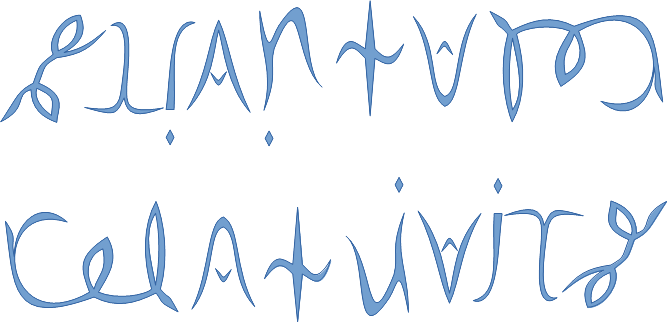See SRQM: QM from SR - The 4-Vector RoadMap (.html)
See SRQM: QM from SR - The 4-Vector RoadMap (.pdf)

SRQM Physics Diagramming Method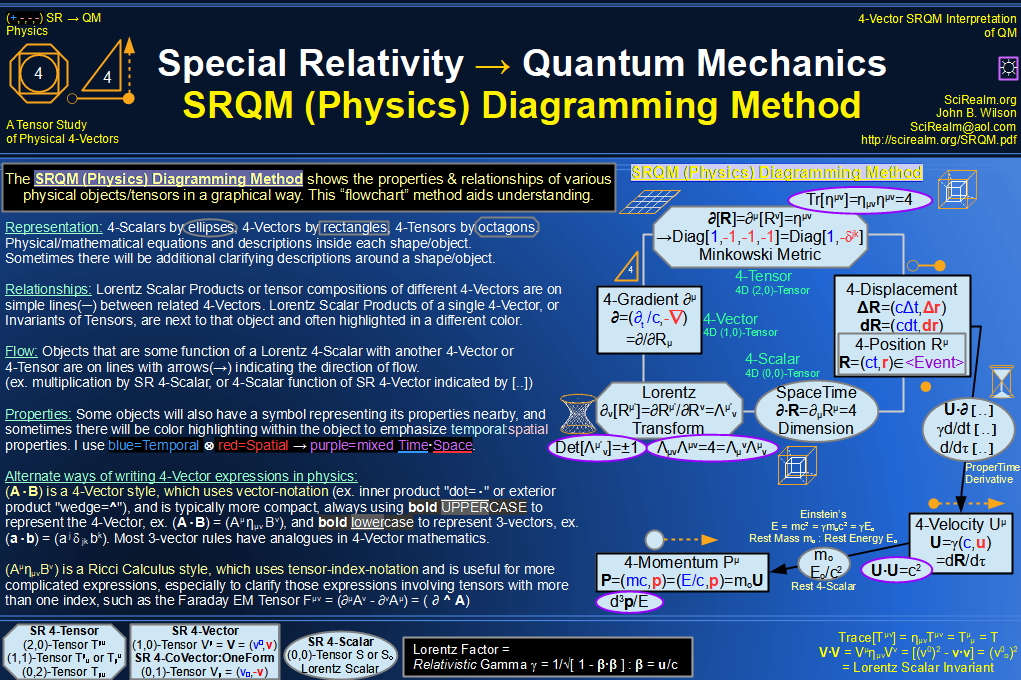SRQM 4-Vector : Four-Vector and Lorentz Scalar DiagramSRQM + EM 4-Vector : Four-Vector and Lorentz Scalar Diagram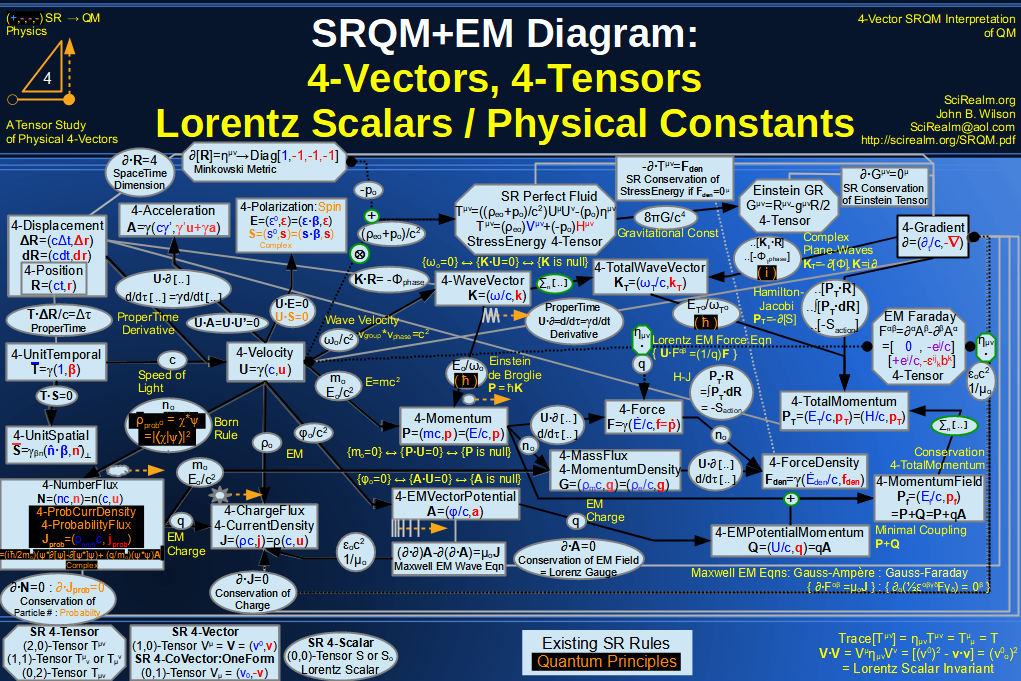SRQM + EM 4-Vector : Four-Vector and Lorentz Scalar Diagram With Tensor Invariants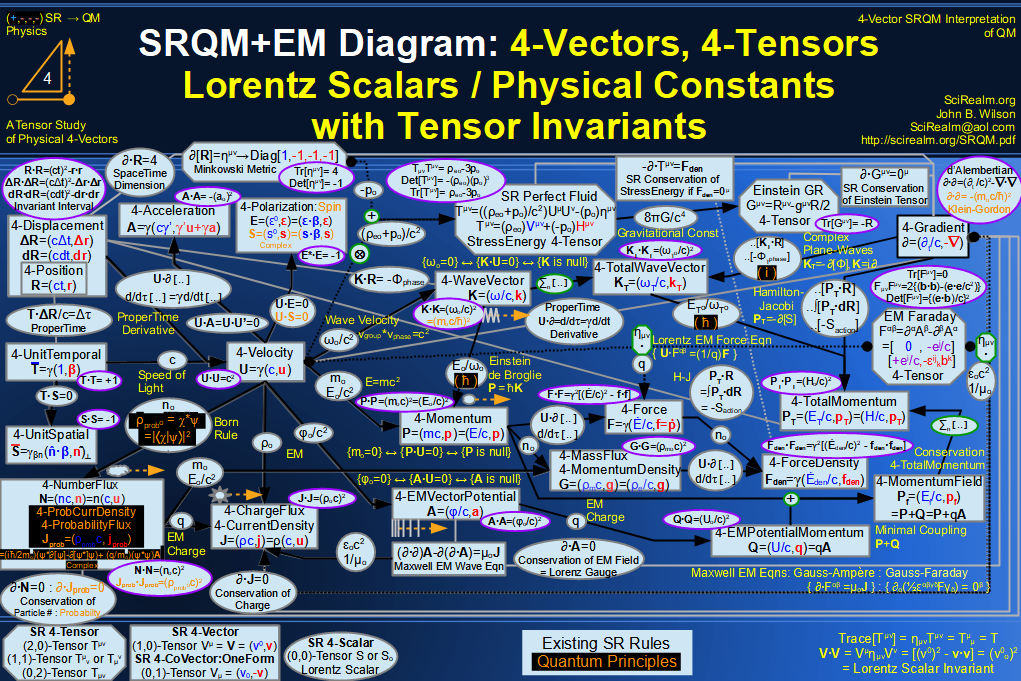SRQM 4-Vector : Four-Vector Stress-Energy & Projection Tensors Diagram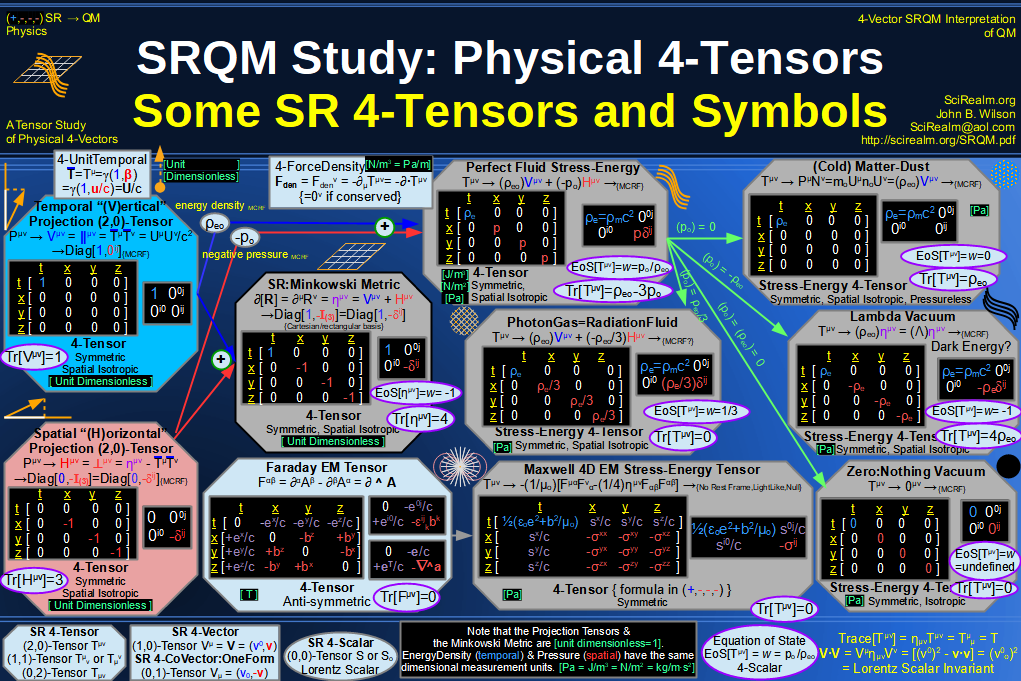SRQM 4-Vector : Four-Vector SR Quantum RoadMapSRQM + EM 4-Vector : Four-Vector SR Quantum RoadMap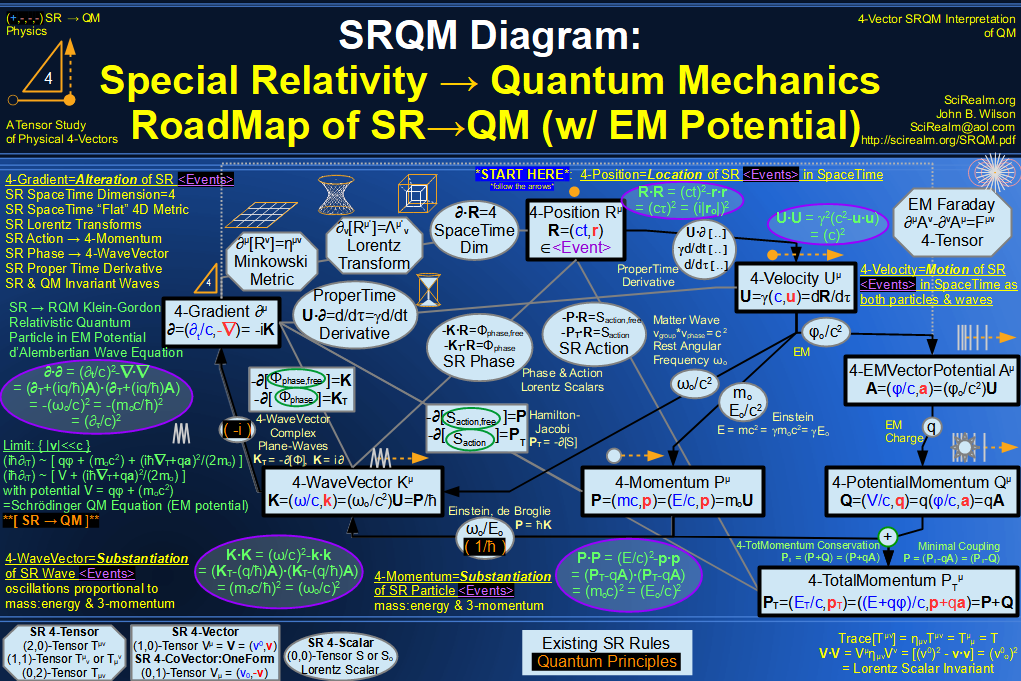SRQM + EM 4-Vector : Four-Vector SR Quantum RoadMap - Simple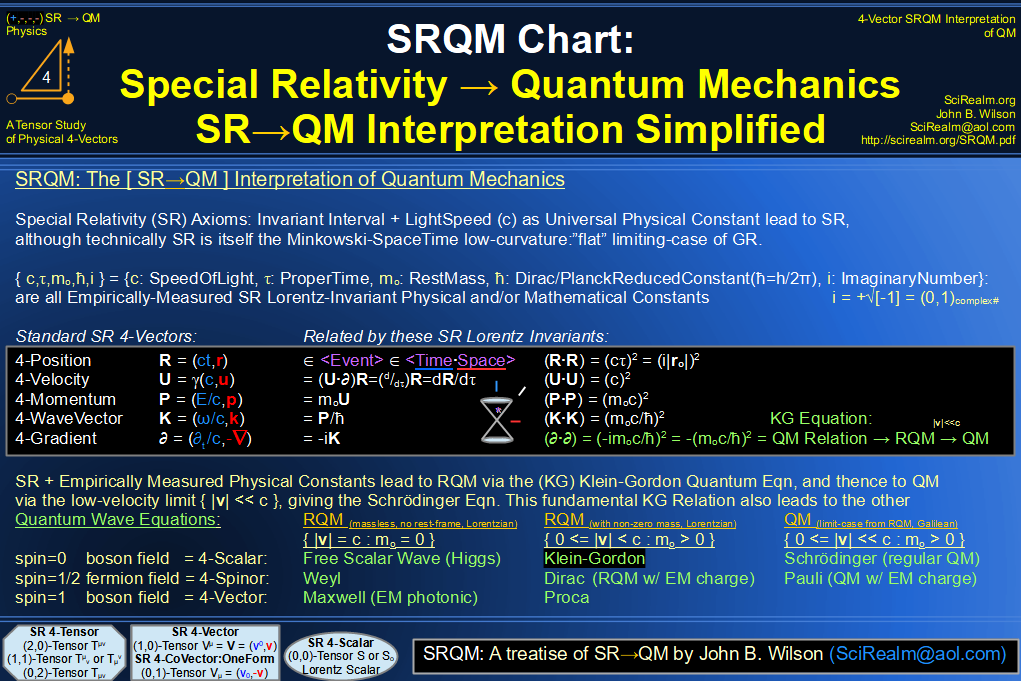SRQM 4-Vector : Four-Vector New Relativistic Quantum ParadigmSRQM + EM 4-Vector : Four-Vector New Relativistic Quantum Paradigm (with EM)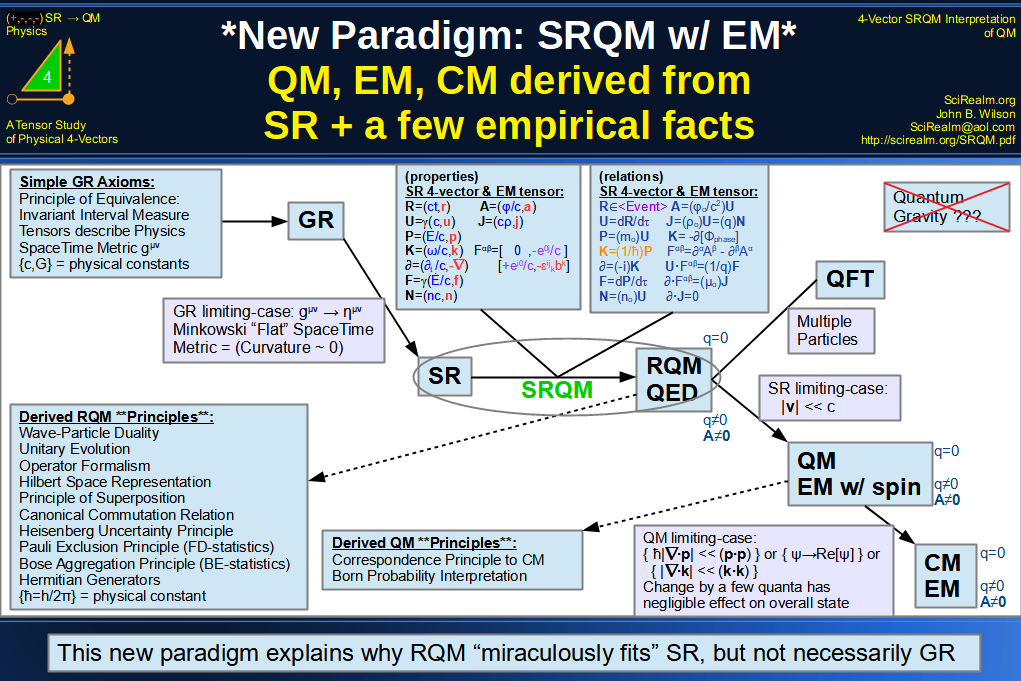SRQM 4-Vector : Four-Vector New Relativistic Quantum Paradigm - Venn DiagramSRQM 4-Vector : Four-Vector SpaceTime is 4D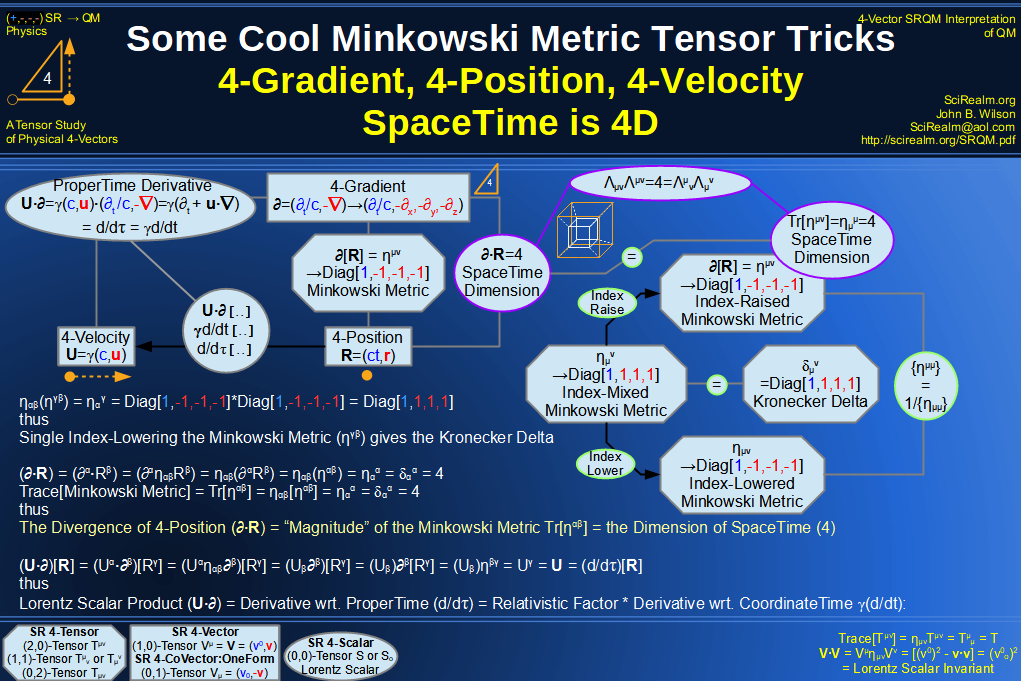SRQM 4-Vector : Four-Vector SpaceTime Orthogonality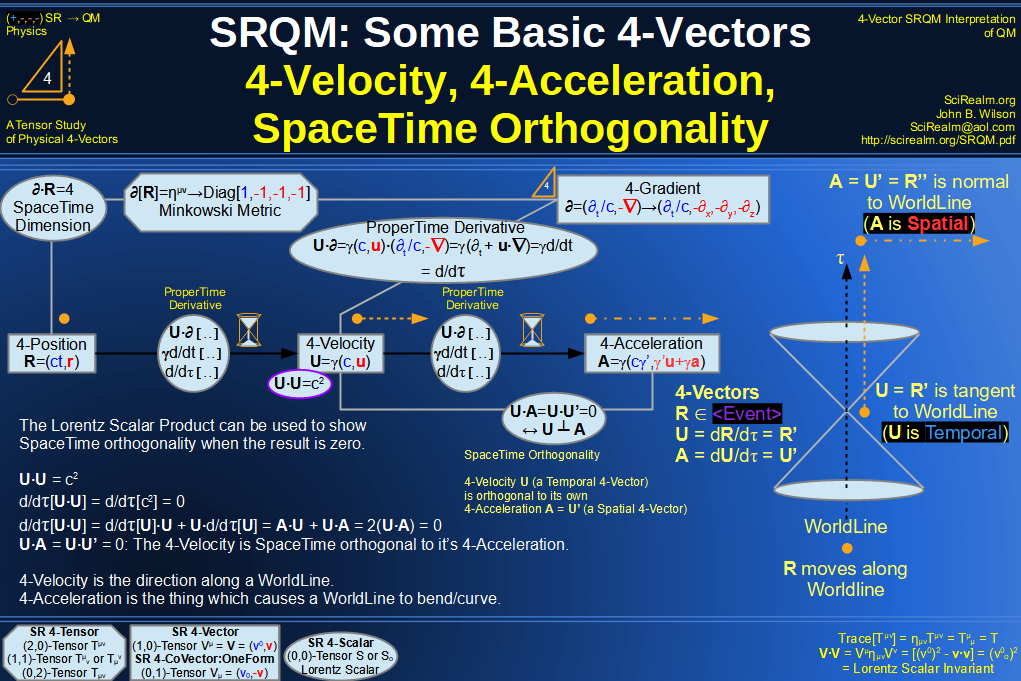SRQM 4-Vector : Four-Vector 4-Position, 4-Velocity, 4-Acceleration Diagram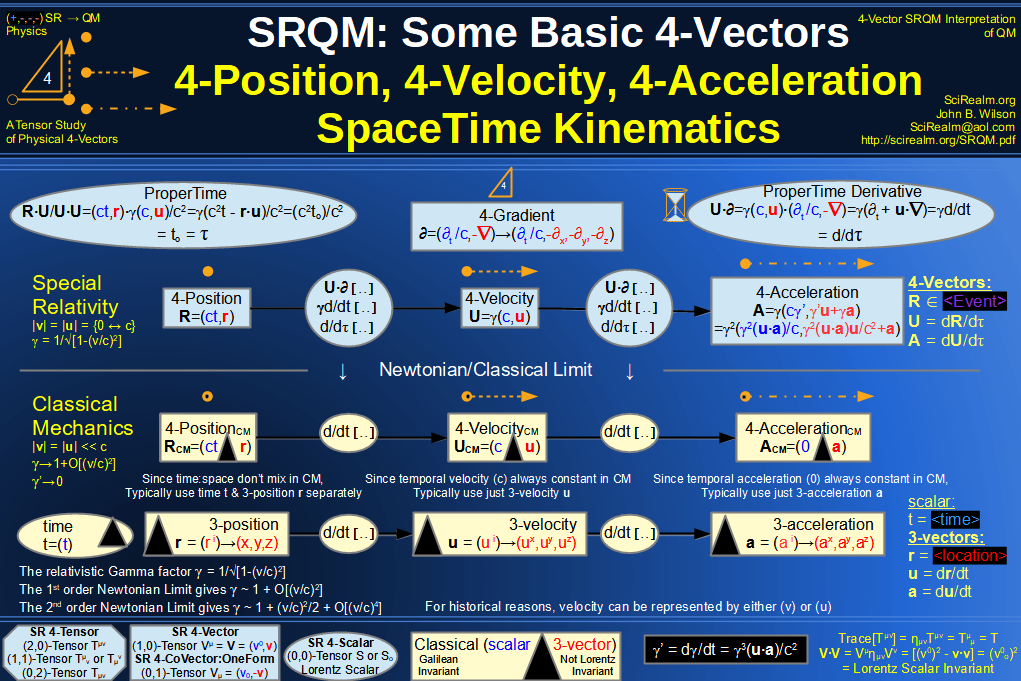SRQM 4-Vector : Four-Vector 4-Displacement, 4-Velocity, Relativity of Simultaneity Diagram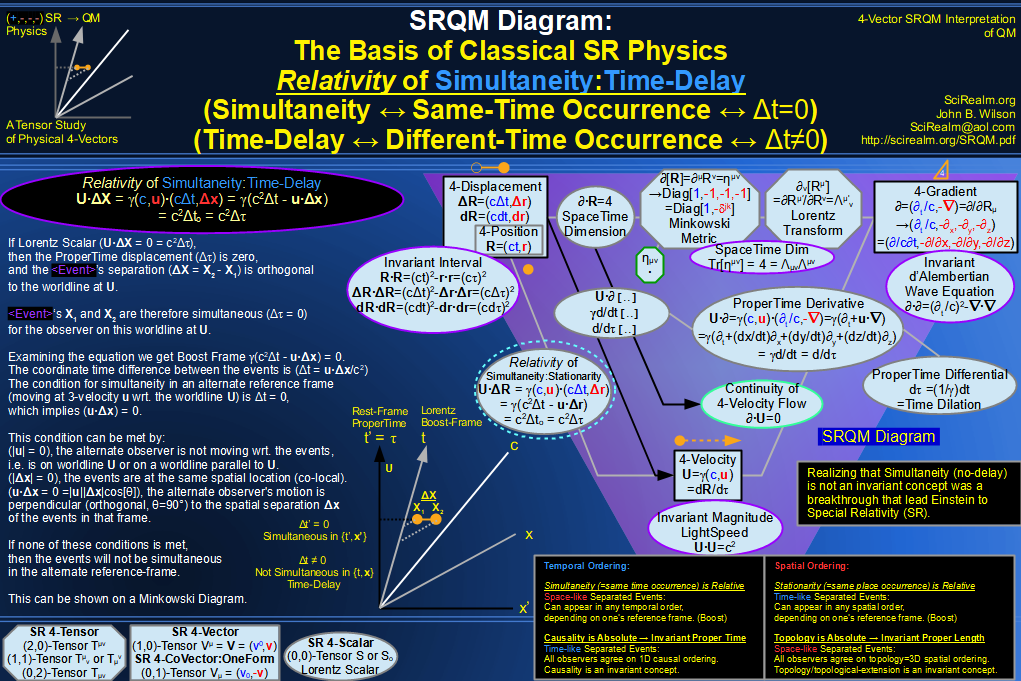SRQM 4-Vector : Four-Vector 4-Velocity, 4-Gradient, Time Dilation Diagram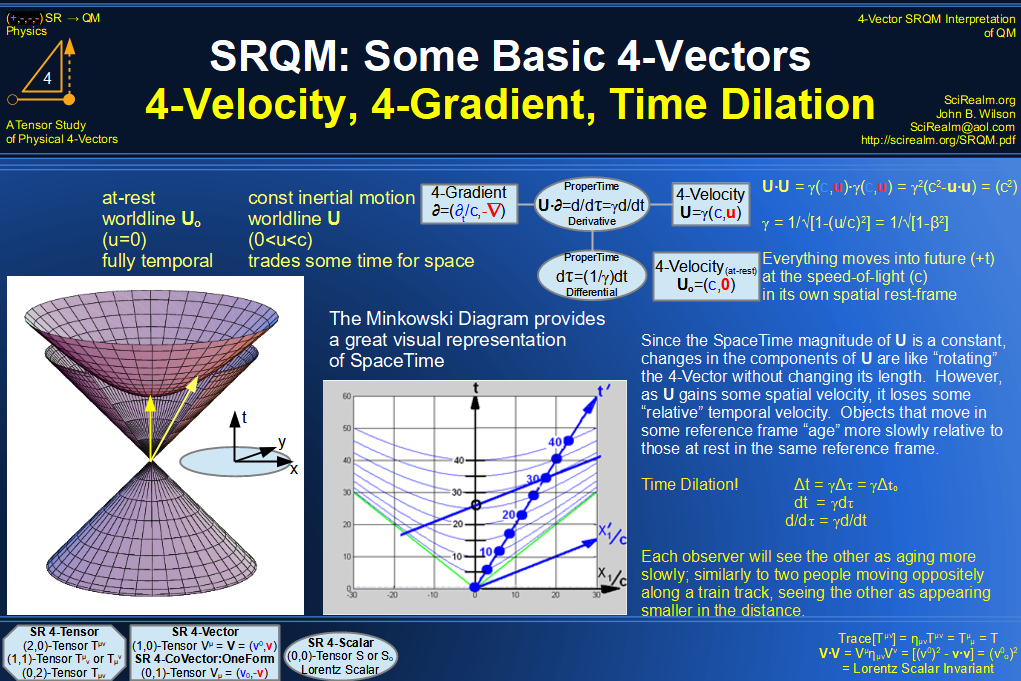SRQM 4-Vector : Four-Vector 4-Vector, 4-Velocity, 4-Momentum, E=mc^2 Diagram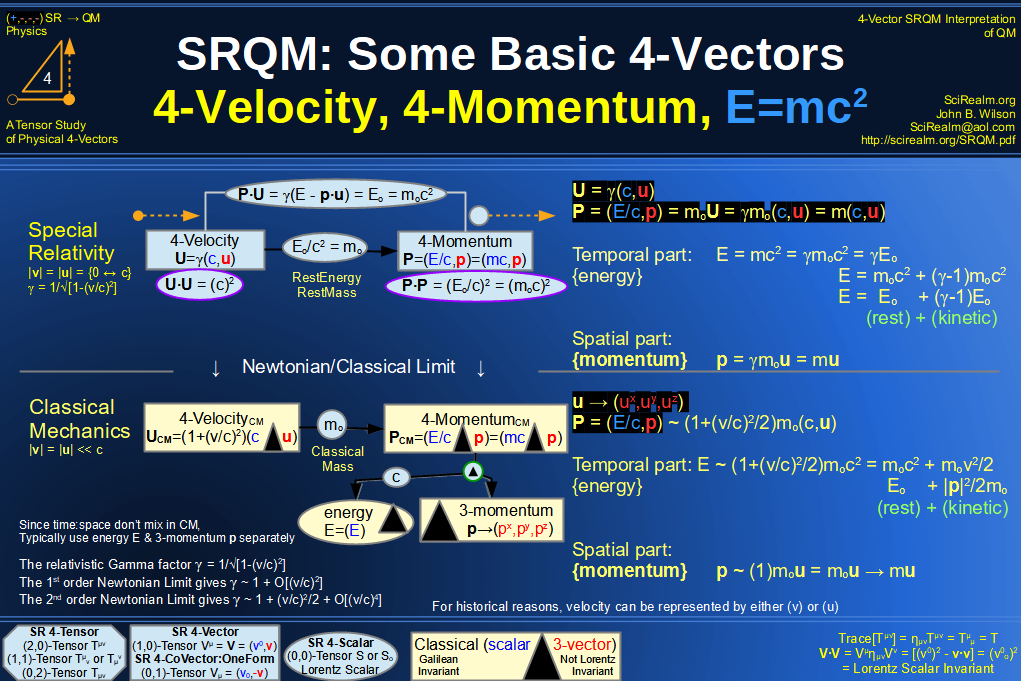SRQM 4-Vector : Four-Vector 4-Velocity, 4-WaveVector, Relativistic Doppler Effect Diagram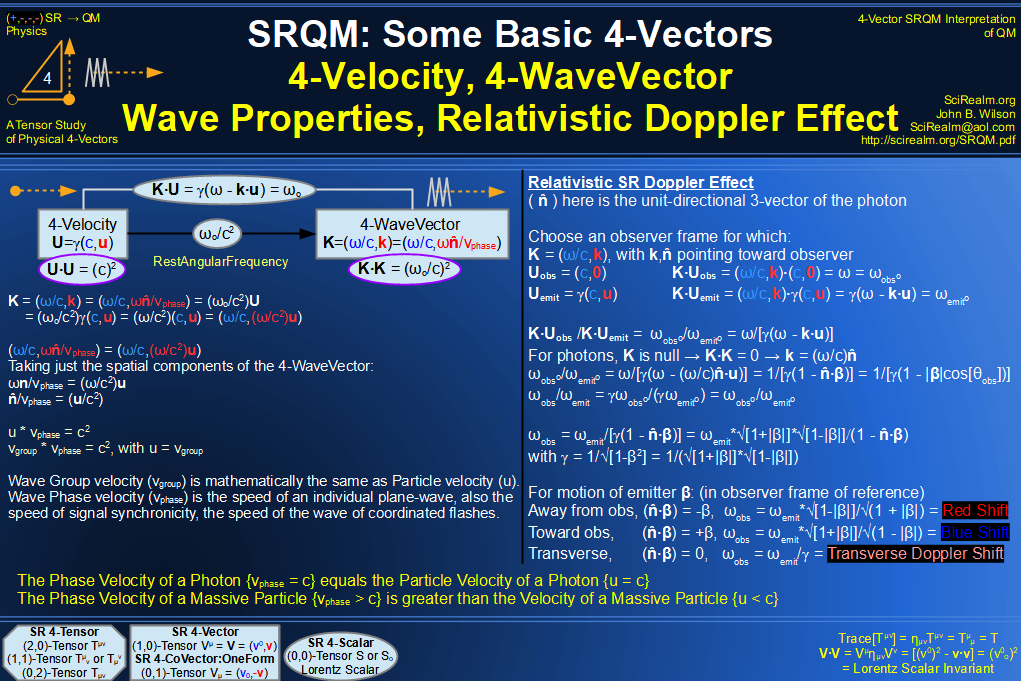SRQM 4-Vector : Four-Vector Wave-Particle Diagram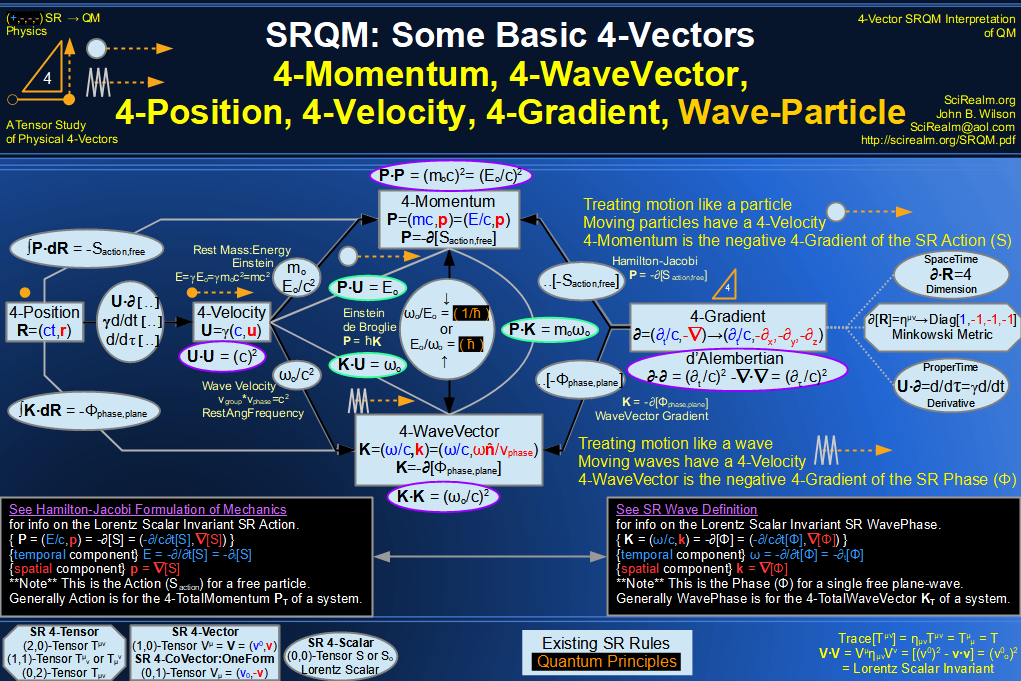SRQM 4-Vector : Four-Vector Compton Effect Diagram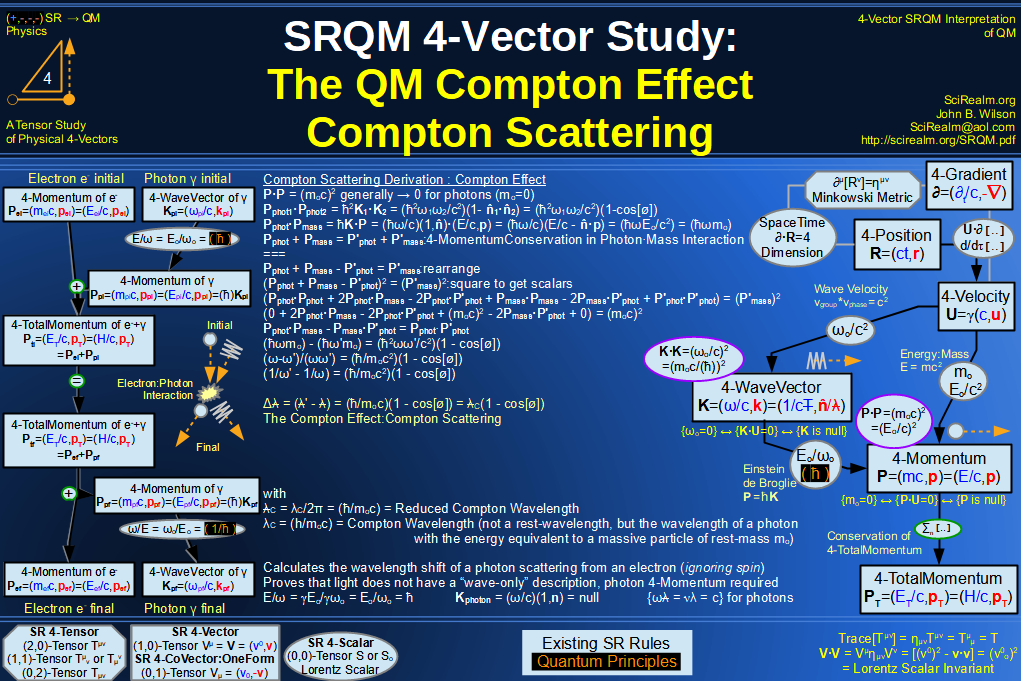SRQM 4-Vector : Four-Vector Aharonov-Bohm Effect Diagram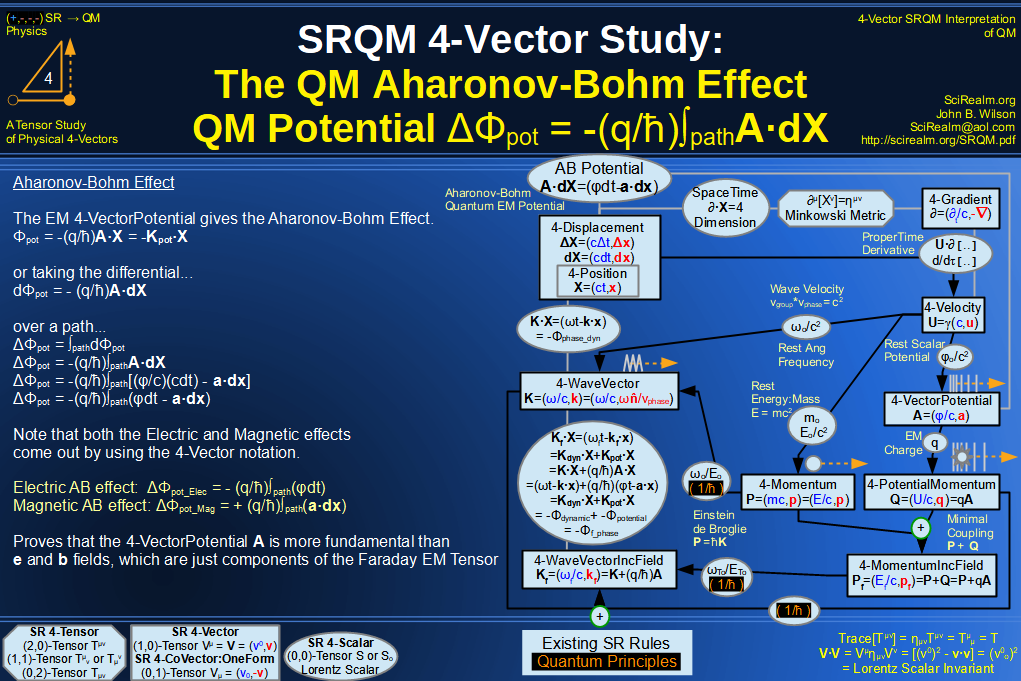SRQM 4-Vector : Four-Vector Josephson Junction Effect Diagram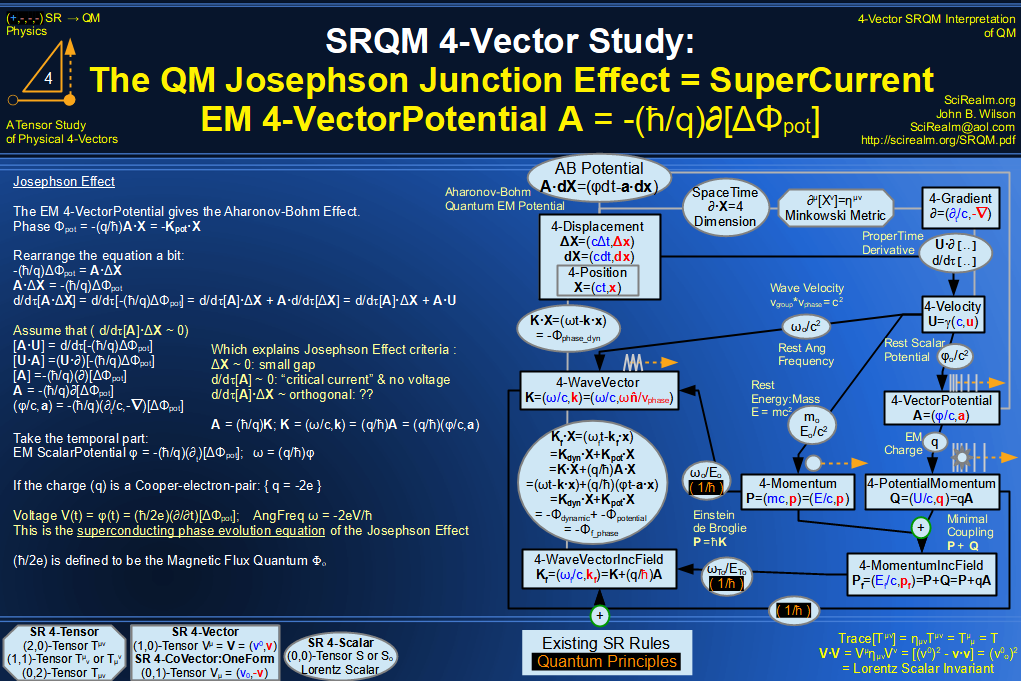SRQM 4-Vector : Four-Vector Hamilton-Jacobi vs Action, Josephson vs Aharonov-Bohm Diagram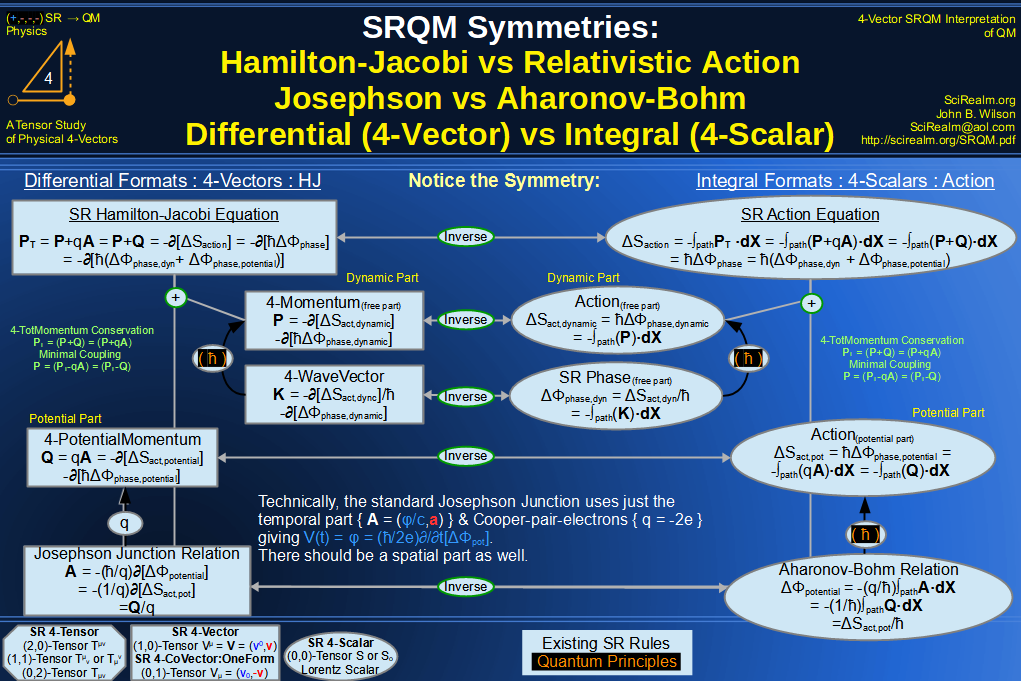SRQM 4-Vector : Four-Vector Motion of Lorentz Scalar Invariants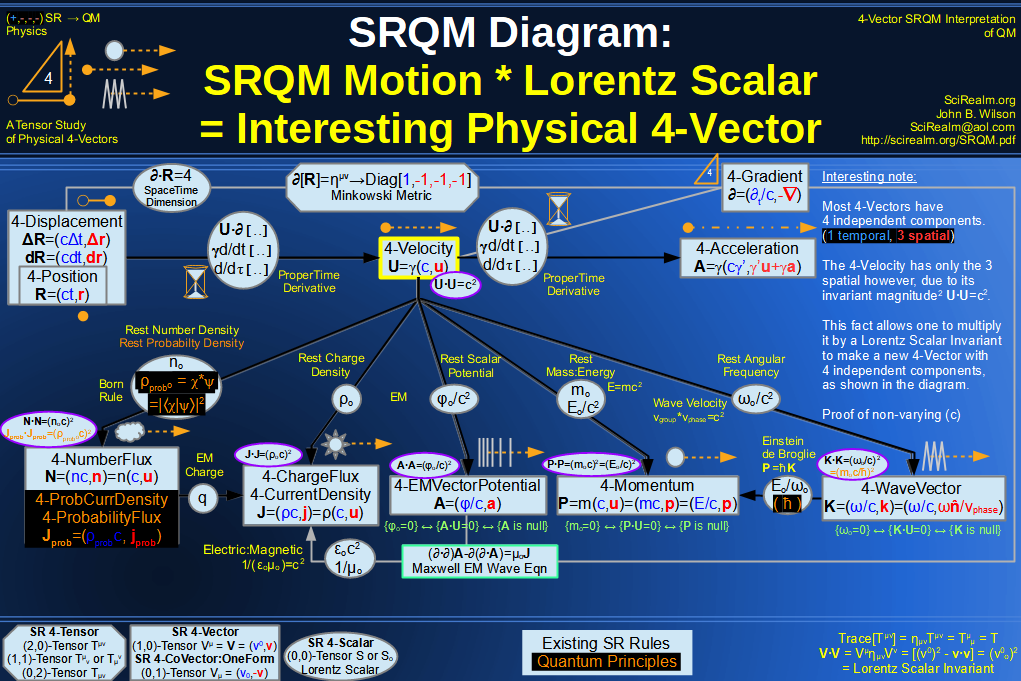SRQM 4-Vector : Four-Vector Motion of Lorentz Scalar Invariants, Conservation Laws & Continuity Equations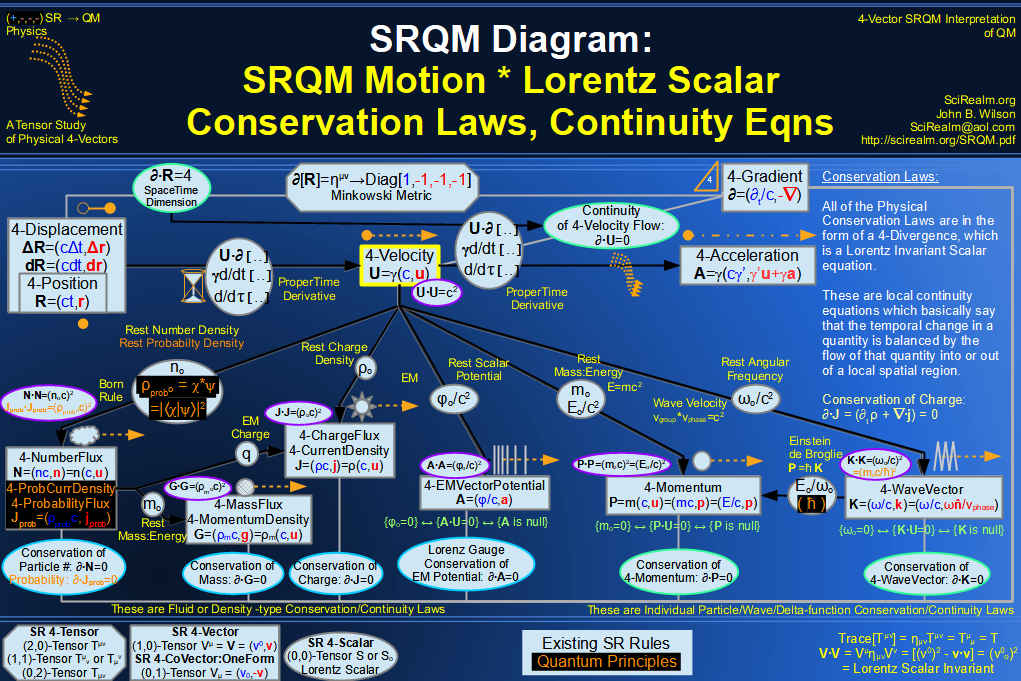SRQM 4-Vector : Four-Vector Speed of Light (c)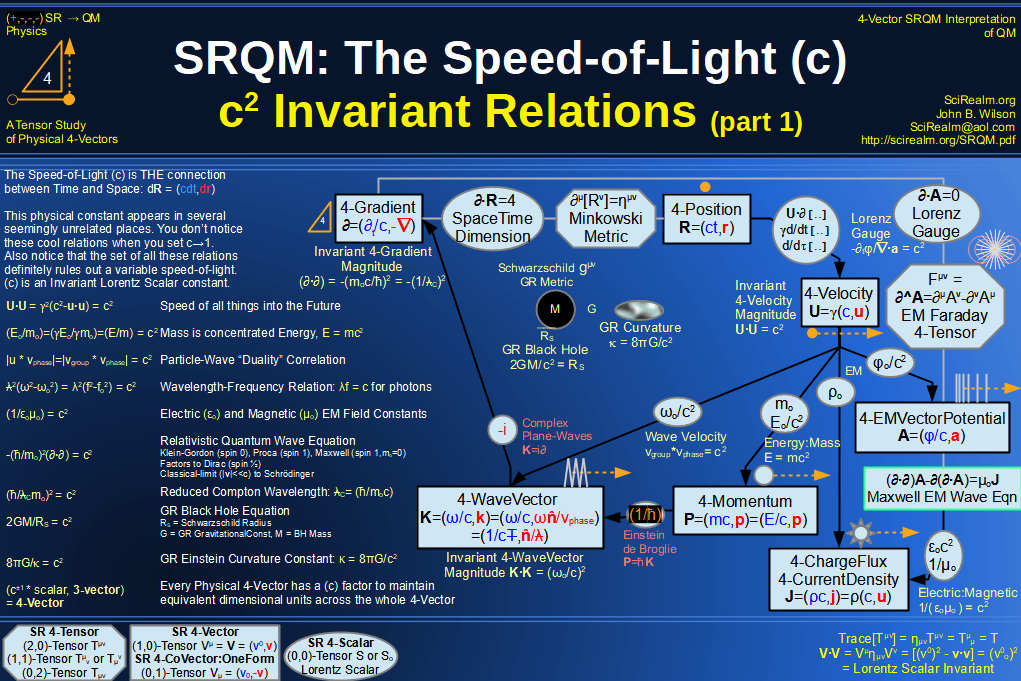SRQM 4-Vector : Four-Vector Minimal Coupling Conservation of 4-TotalMomentum)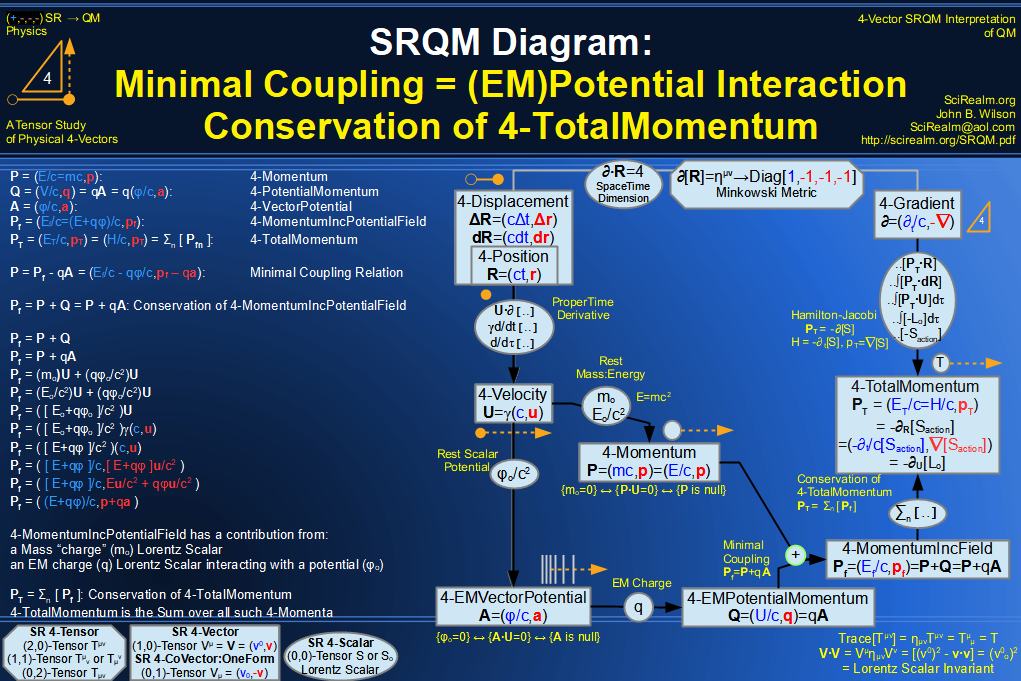SRQM 4-Vector : Four-Vector Relativistic Action (S) Diagram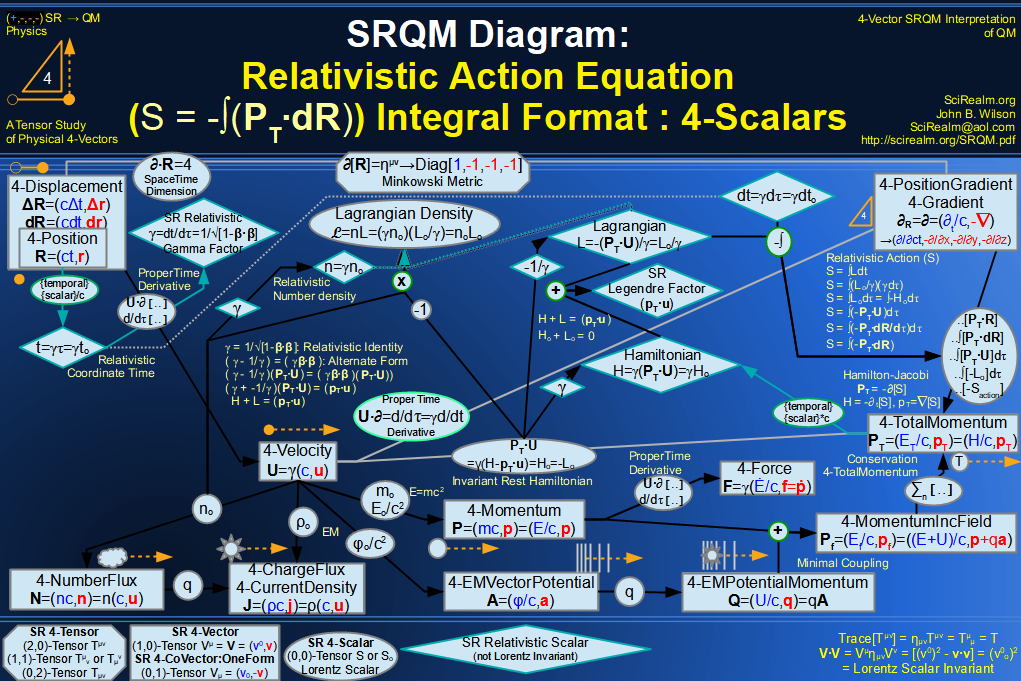SRQM 4-Vector : Four-Vector Relativistic Lagrangian Hamiltonian Diagram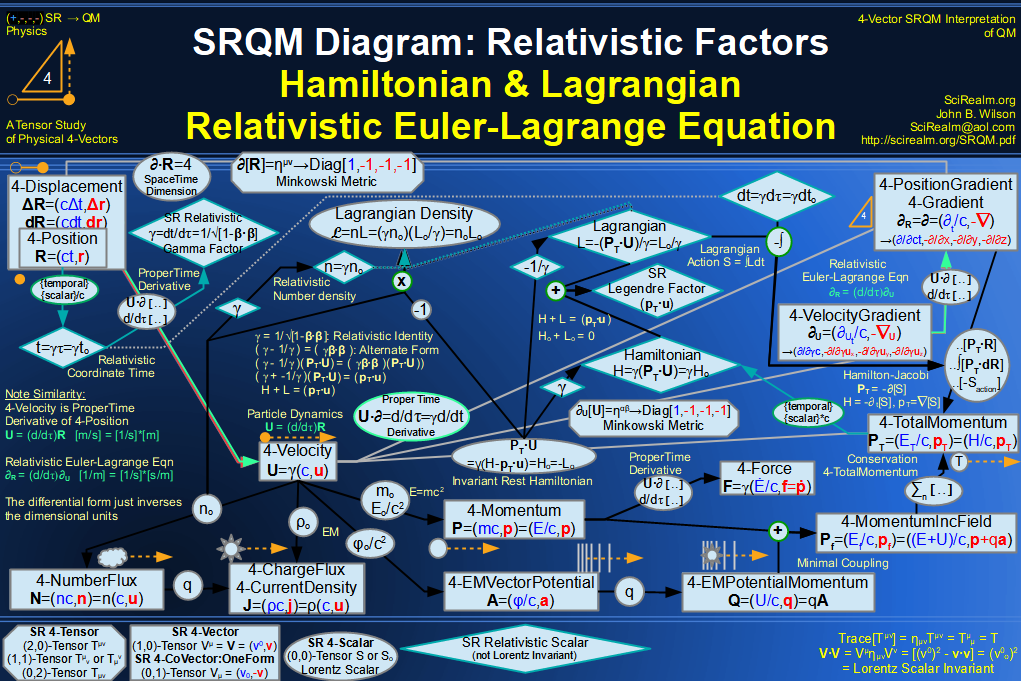SRQM 4-Vector : Four-Vector Relativistic Euler-Lagrange Equation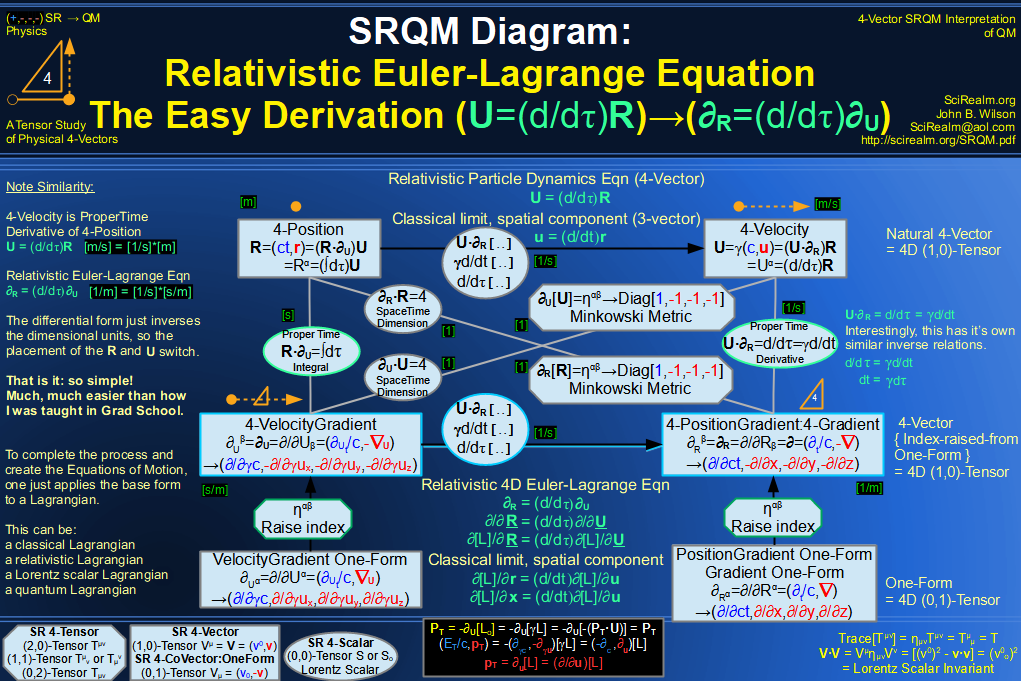SRQM 4-Vector : Four-Vector Relativistic EM Equations of Motion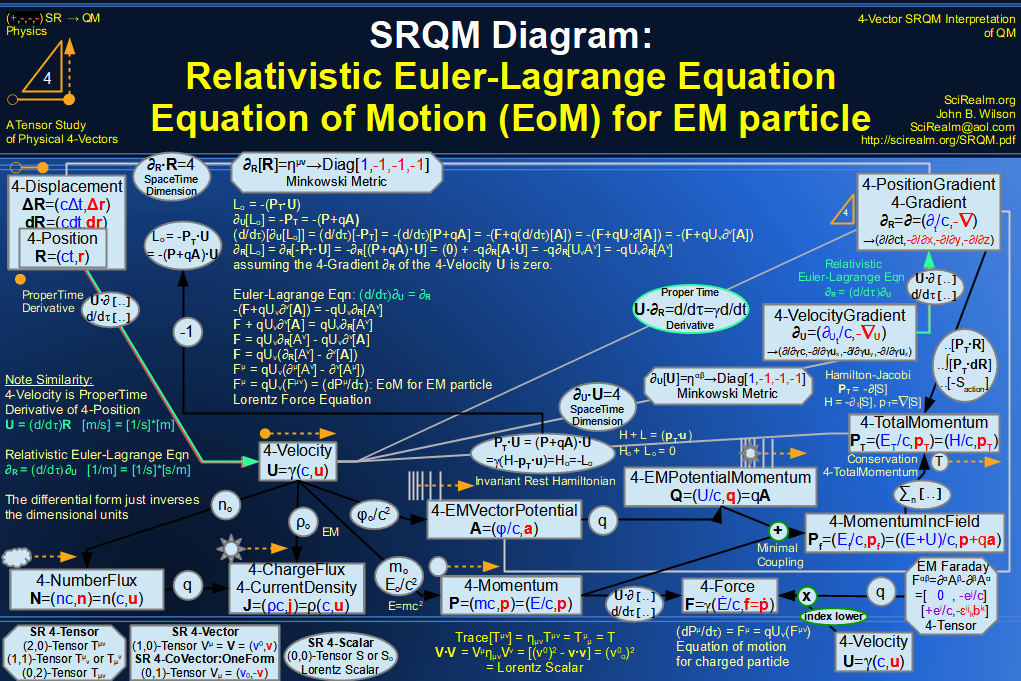SRQM 4-Vector : Four-Vector Einstein-de Broglie Relation hbar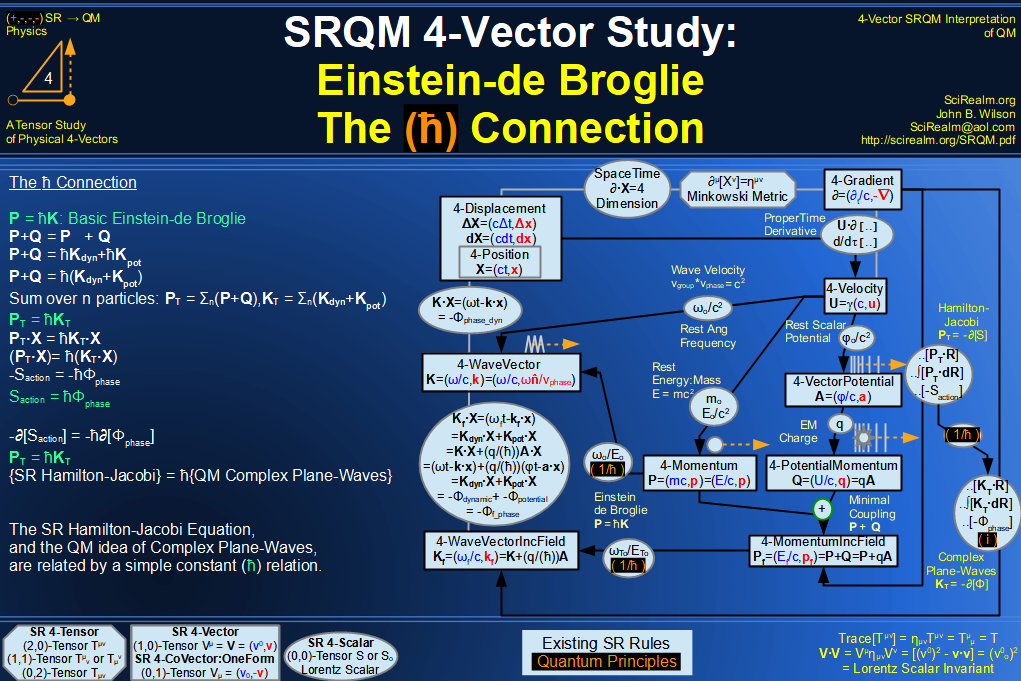SRQM 4-Vector : Four-Vector Quantum Canonical Commutation Relation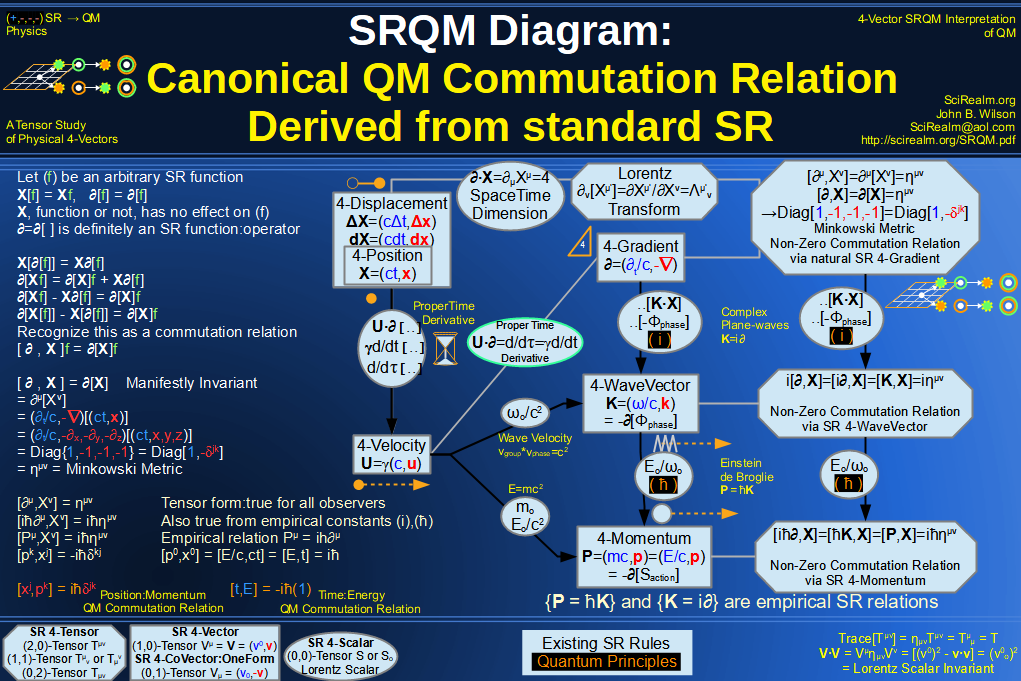SRQM 4-Vector : Four-Vector QM Schroedinger Relation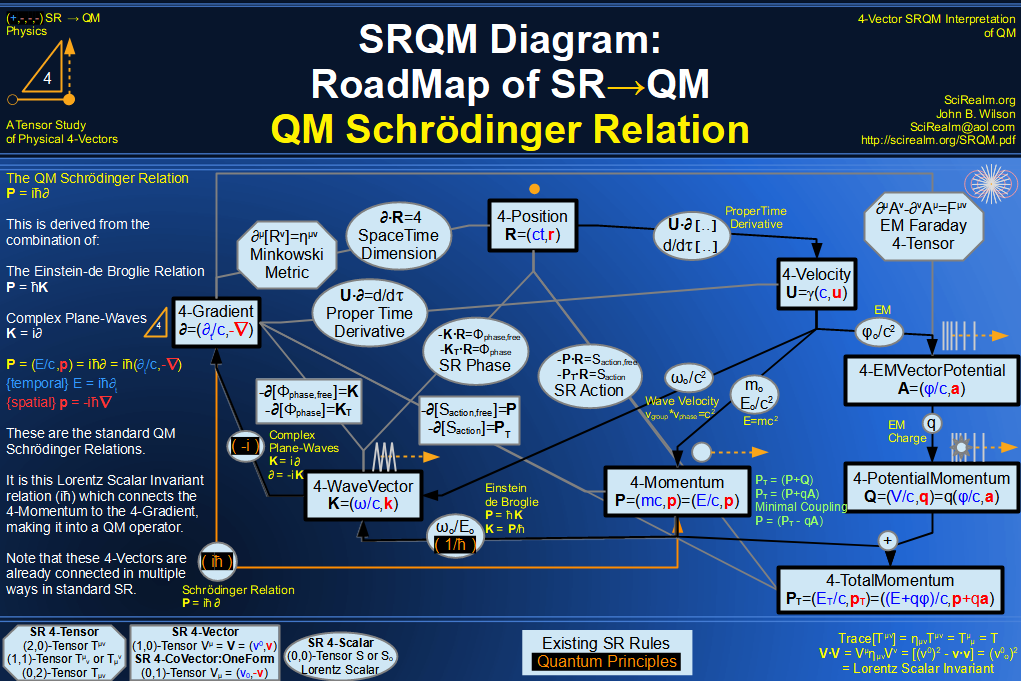SRQM 4-Vector : Four-Vector Quantum Probability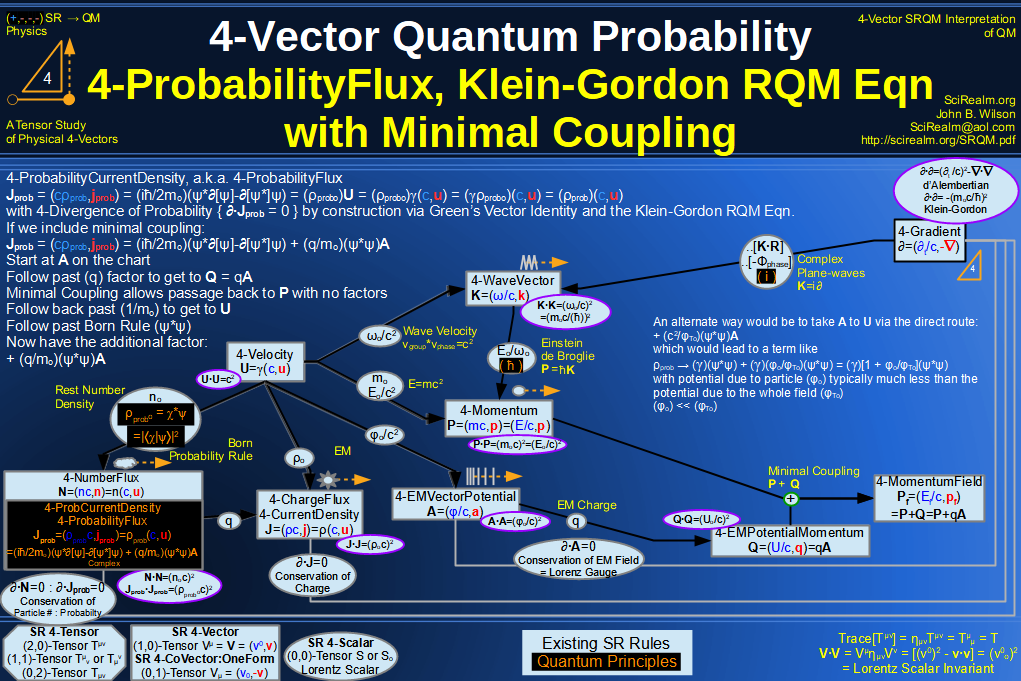SRQM 4-Vector : Four-Vector CPT Theorem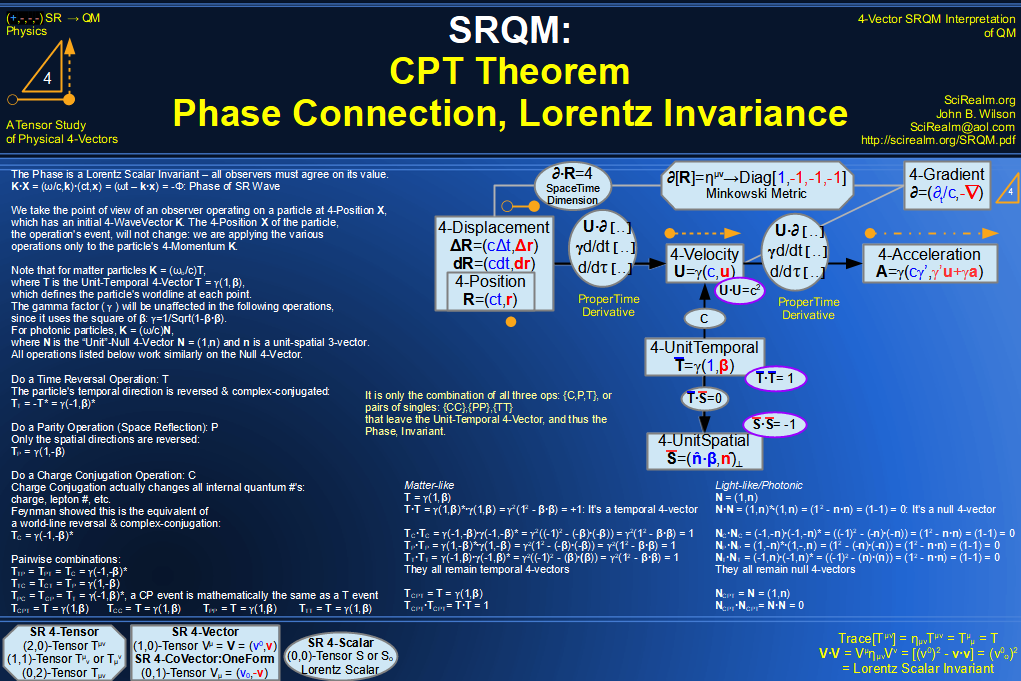SRQM 4-Vector : Four-Vector Lorentz Transforms Connection Map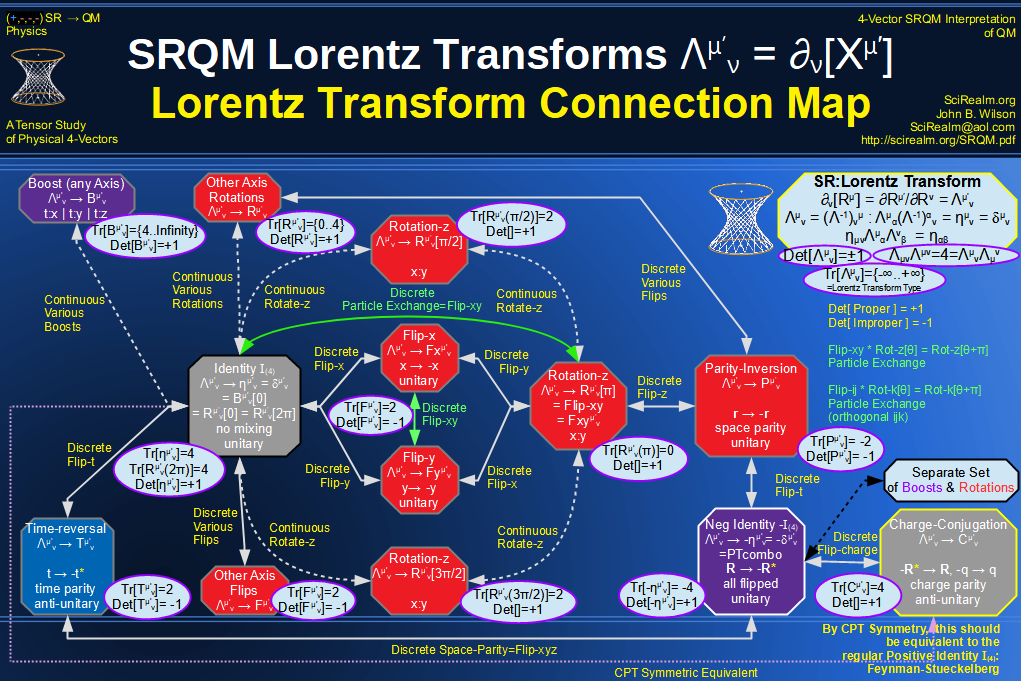SRQM 4-Vector : Four-Vector Lorentz Discrete Transforms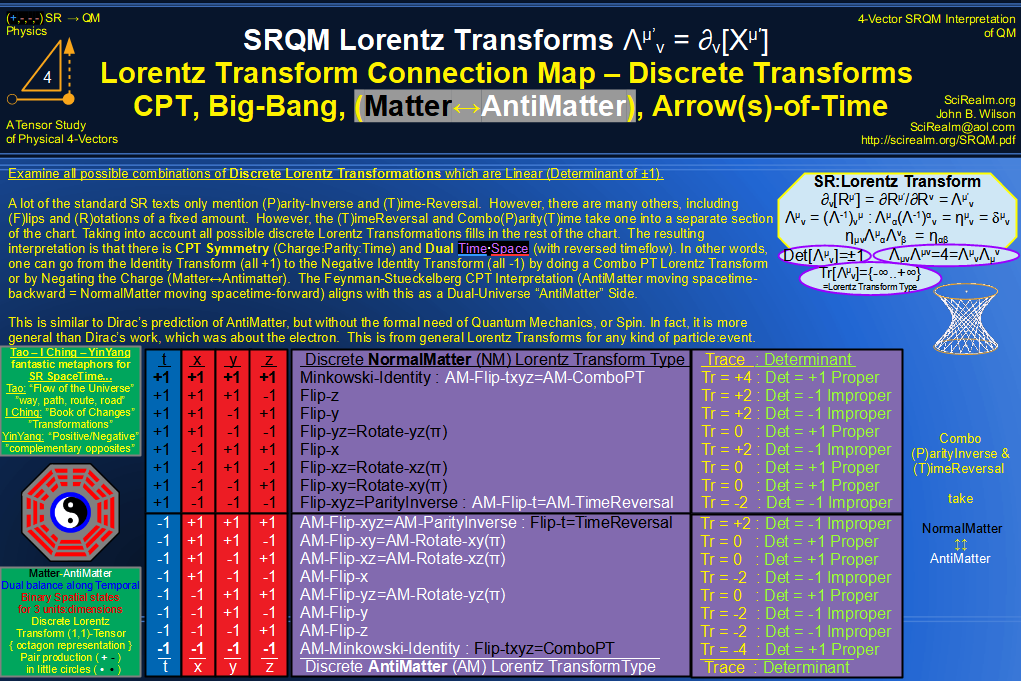SRQM 4-Vector : Four-Vector Lorentz Transforms - Trace Identification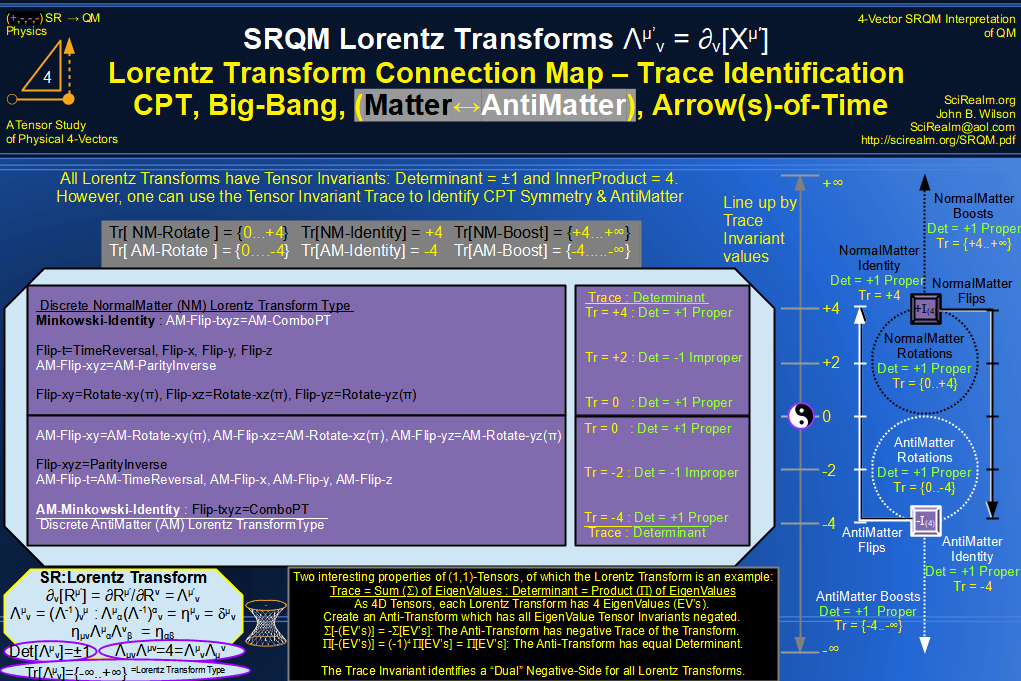SRQM 4-Vector : Four-Vector Lorentz Lorentz Transforms-Interpretations
CPT Symmetry, Baryon Asymmetry Problem Solution, Matter-Antimatter Symmetry Solution, Arrow-of-Time Problem Solution, Big-Bang!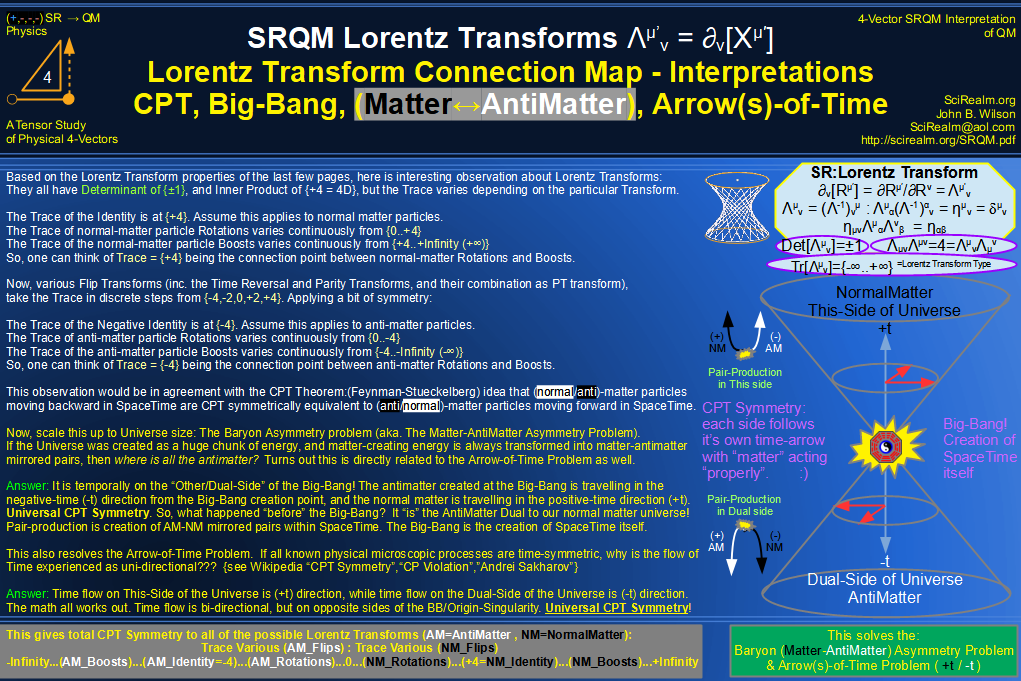See SRQM: QM from SR - The 4-Vector RoadMap (.html)
See SRQM: QM from SR - The 4-Vector RoadMap (.pdf)The Science Realm: John's Virtual Sci-Tech Universe John's Science & Math Stuff: | About John | Send email to John 4-Vectors | Ambigrams | Antipodes | Covert Ops Fragments | Cyrillic Projector | Forced Induction (Sums Of Powers Of Integers) | Fractals | Frontiers | JavaScript Graphics | Kid Science | Kryptos | Philosophy | Photography | Prime Sieve | QM from SR | QM from SR-Simple RoadMap | SR 4-Vector & Tensor Calculator | Quantum Phase | Quotes | RuneQuest Cipher Challenge | Scientific Calculator (complex capable) | Secret Codes & Ciphers | Science+Math | Sci-Pan Pantheist Poems | Stereograms | SuperMagicSqr4x4 | Turkish Grammar | Quantum Mechanics is derivable from Special Relativity See SRQM - QM from SR - Simple RoadMap (.html) See SRQM - QM from SR - Simple RoadMap (.pdf)